# R S Aggarwal Solutions for Class 11 Maths Chapter 2- Relations

R S Aggarwal Solutions are useful resources for students as it helps them in scoring great marks in the examination. The R S Aggarwal Solutions given in this page contains detailed step-by-step explanations to all the problems that come under Chapter 2, Relations, of the Class 11 R S Aggarwal Textbook. This chapter covers the topics including definition of relation, pictorial diagrams, domain, codomain and range of relation.

These solutions are prepared by subject matter experts at BYJU’S, explaining the method of solving problems, right from scratch. By understanding the concepts used in R S Aggarwal Solutions for Class 11 Maths, students will be able to clear all their doubts related to Relations.

### Download PDF of R S Aggarwal Solutions for Class 11 Maths Chapter 2- Relations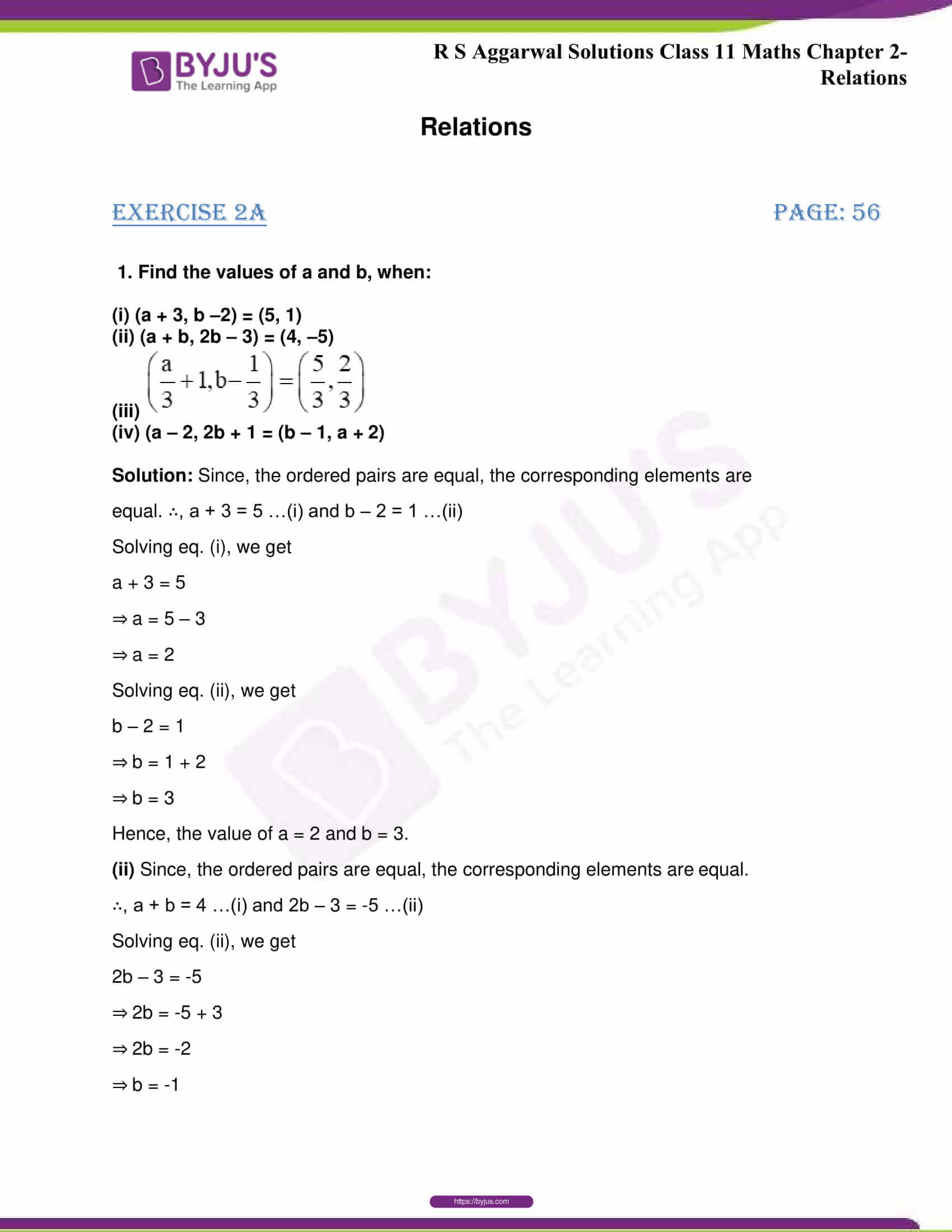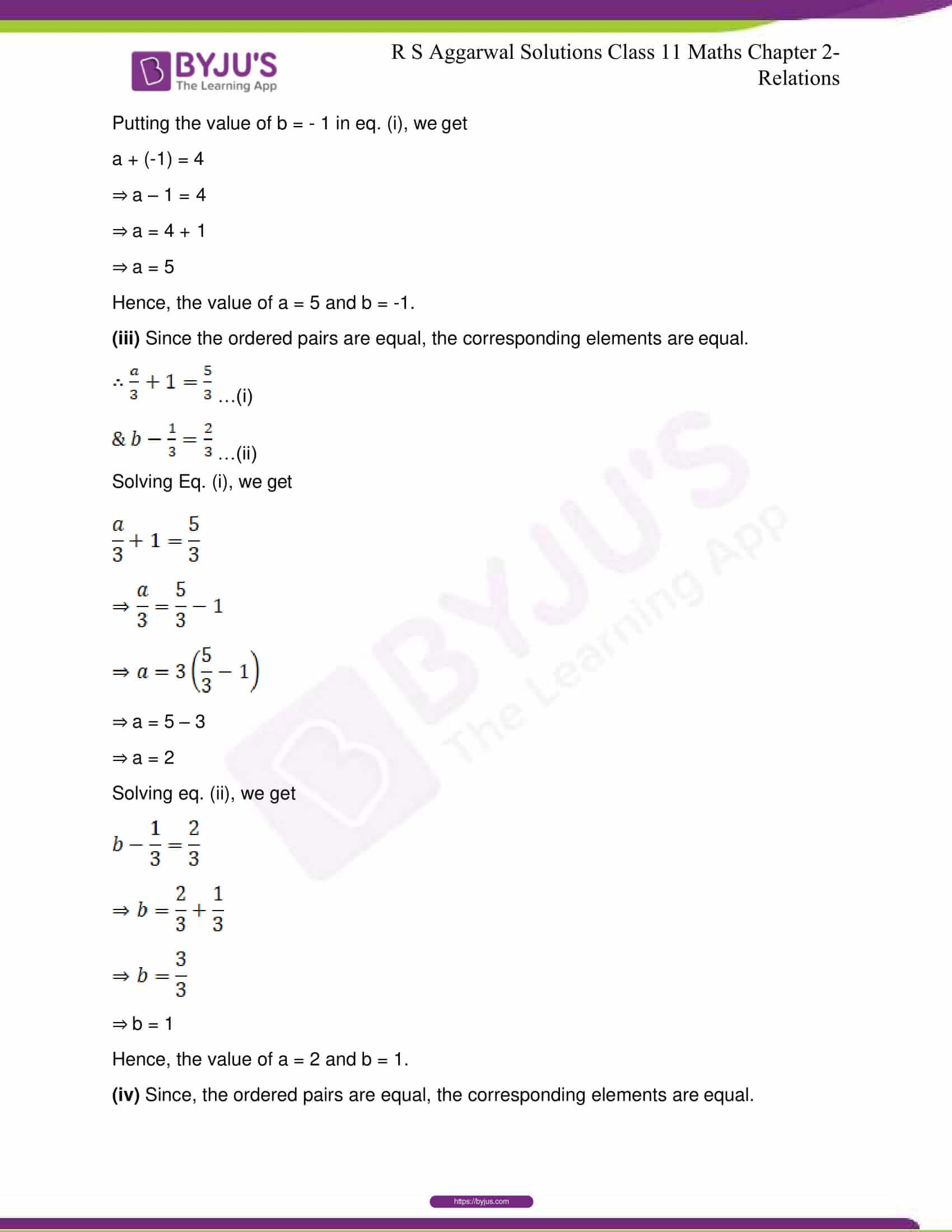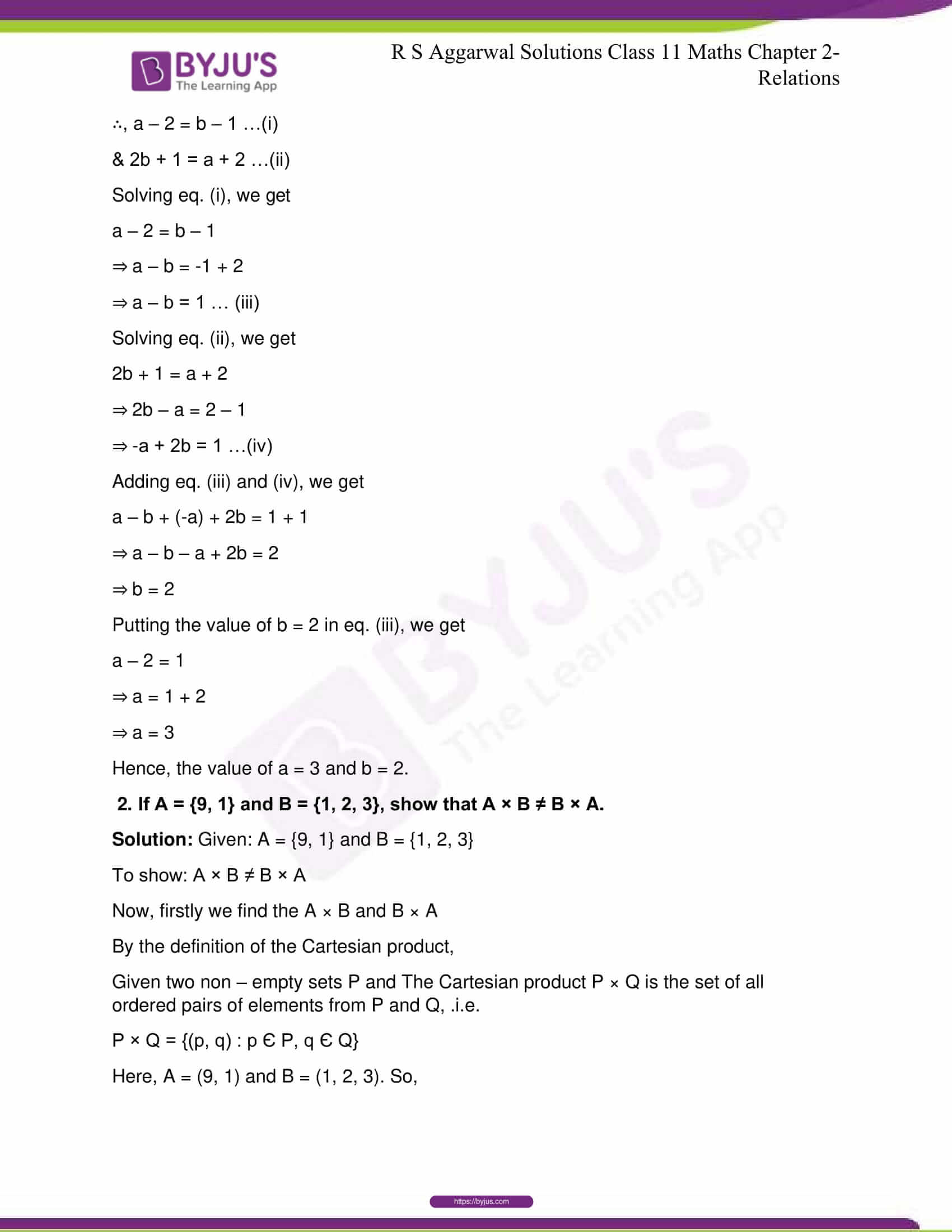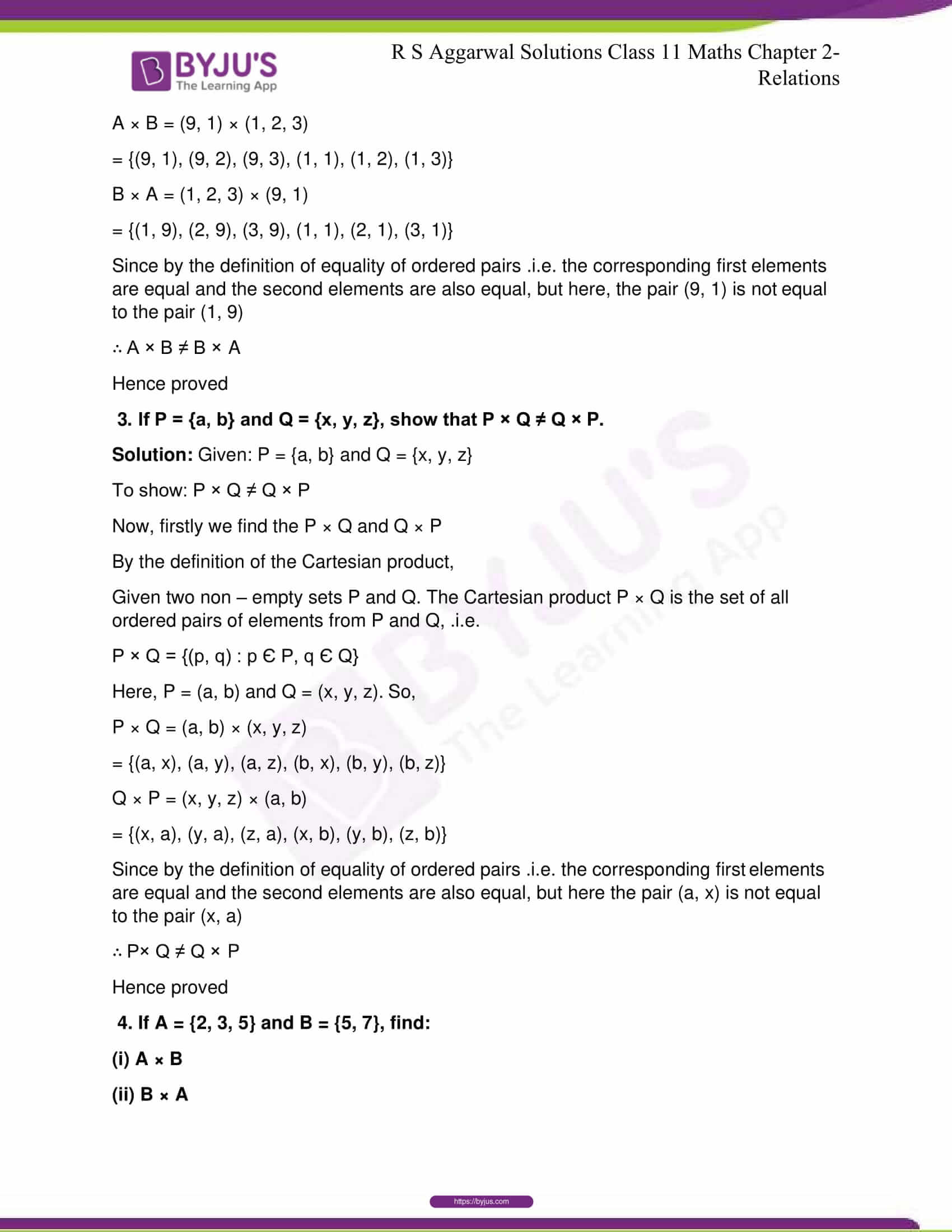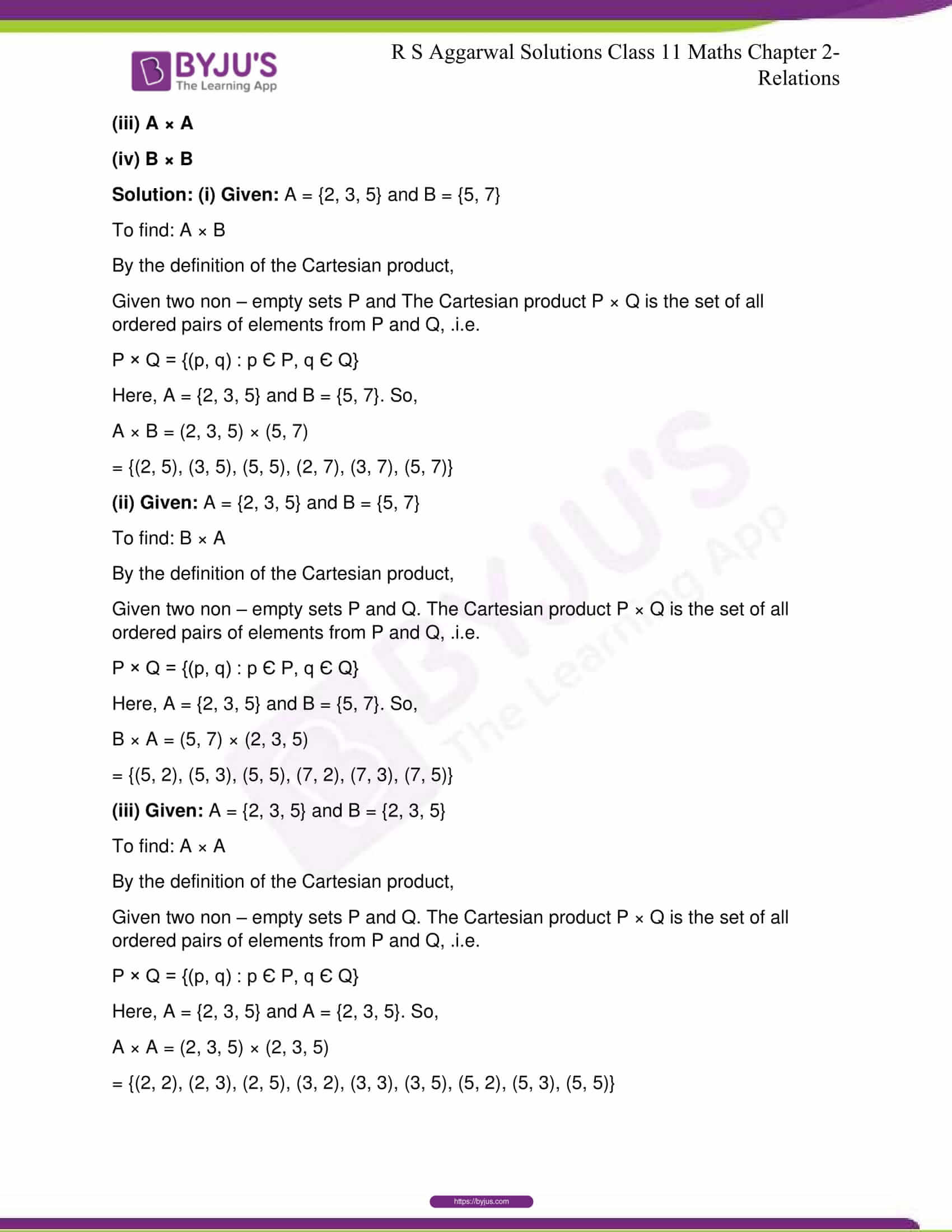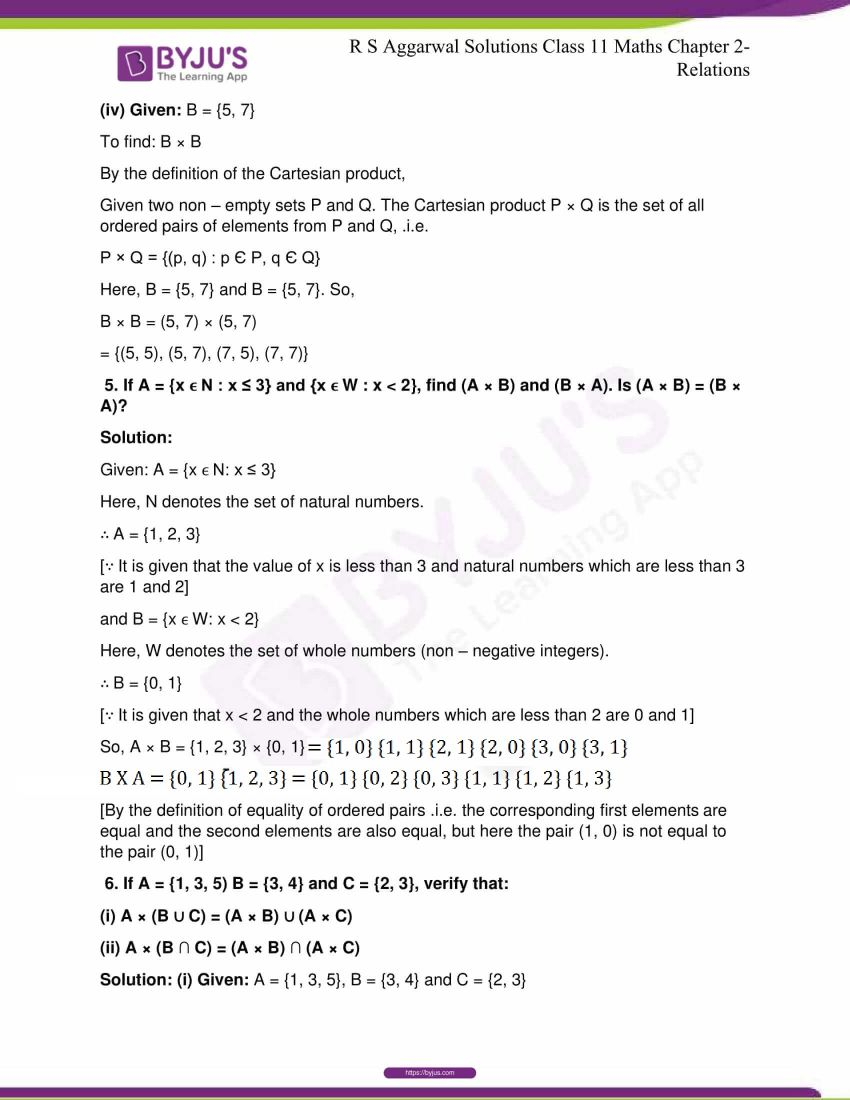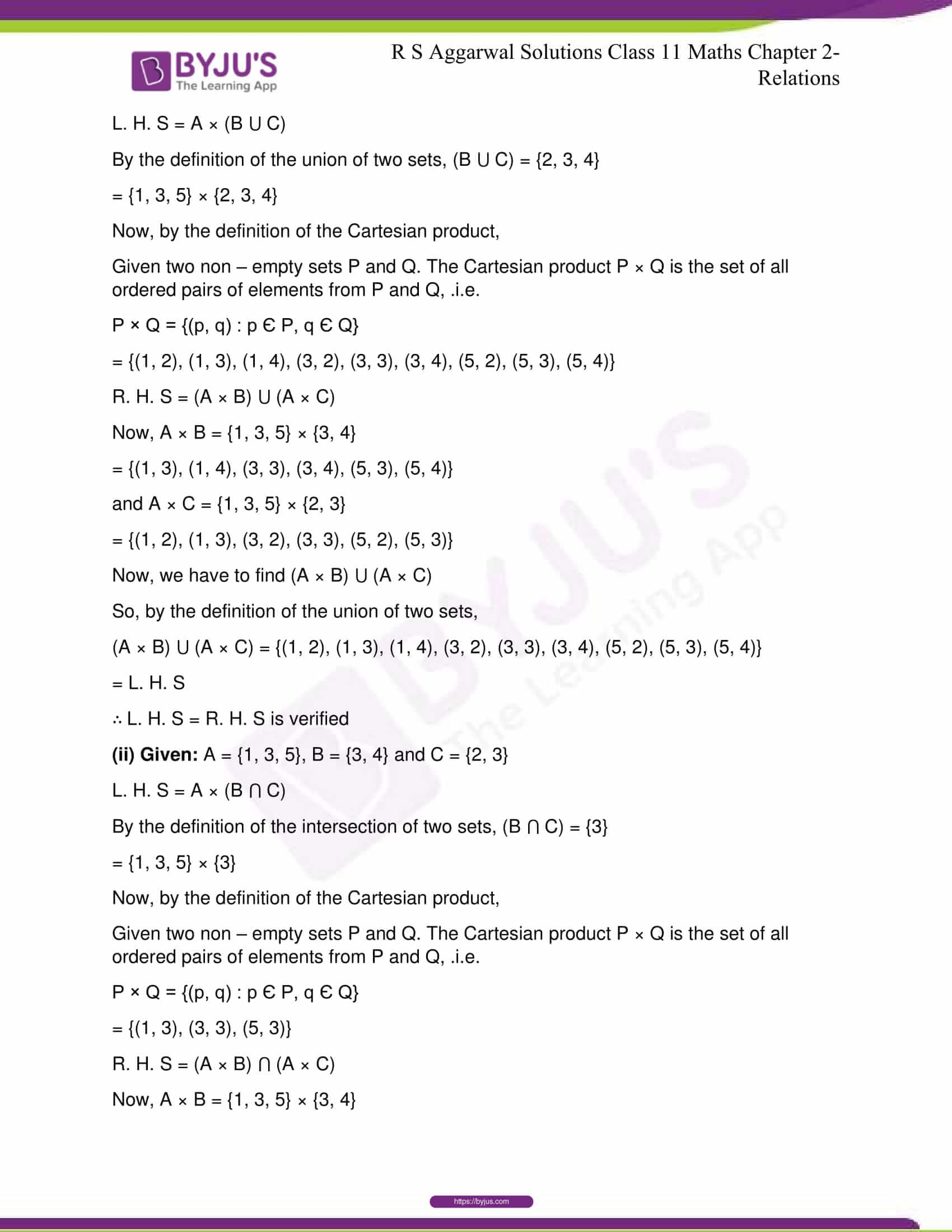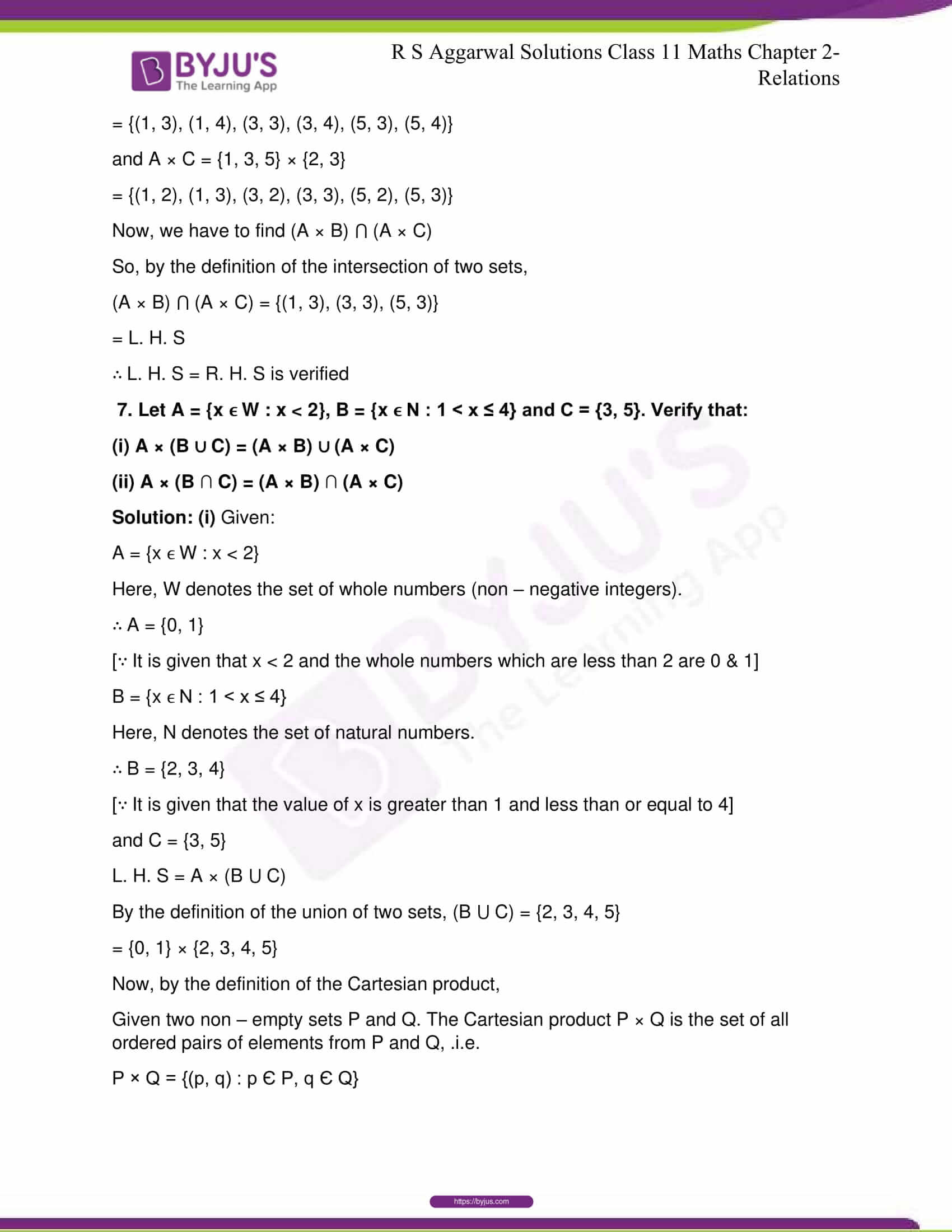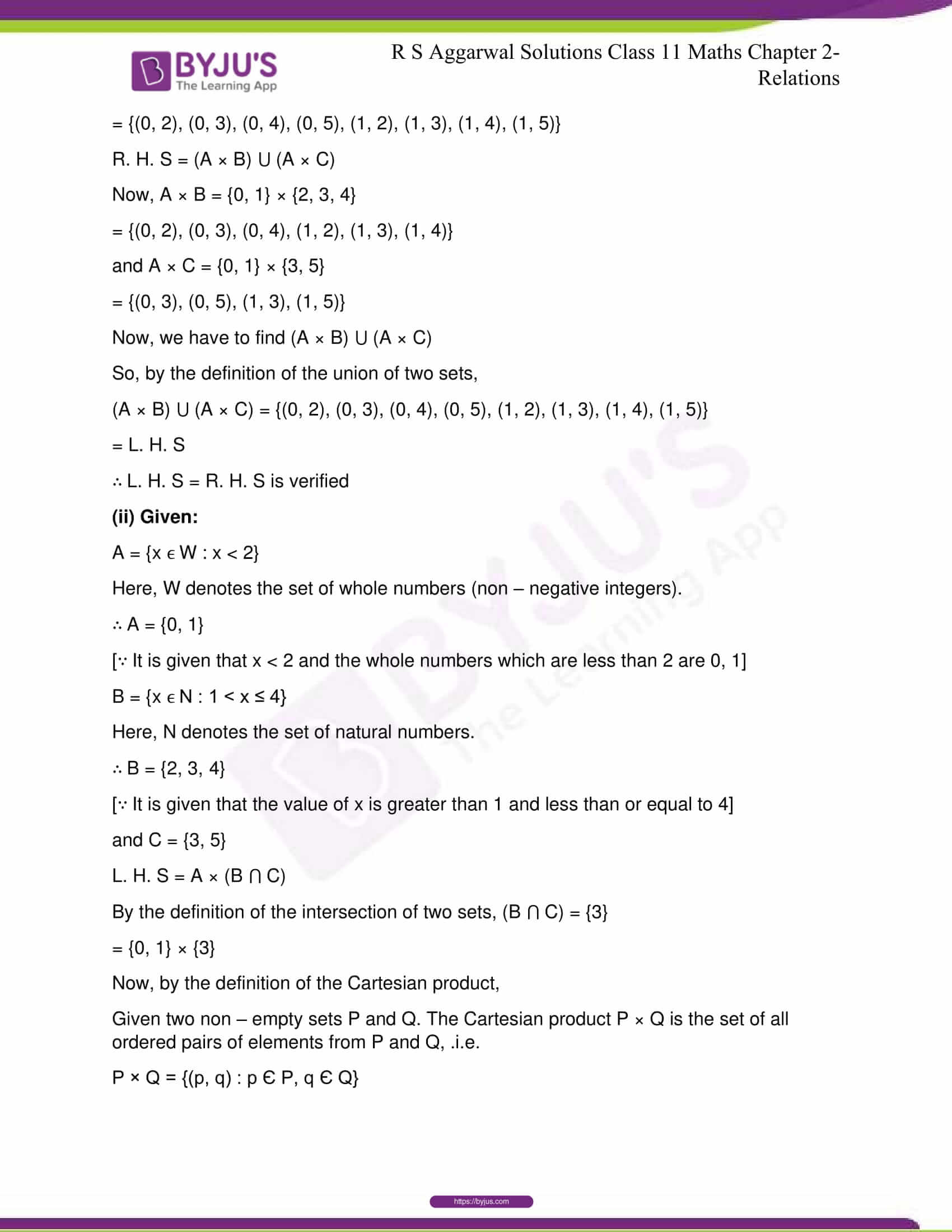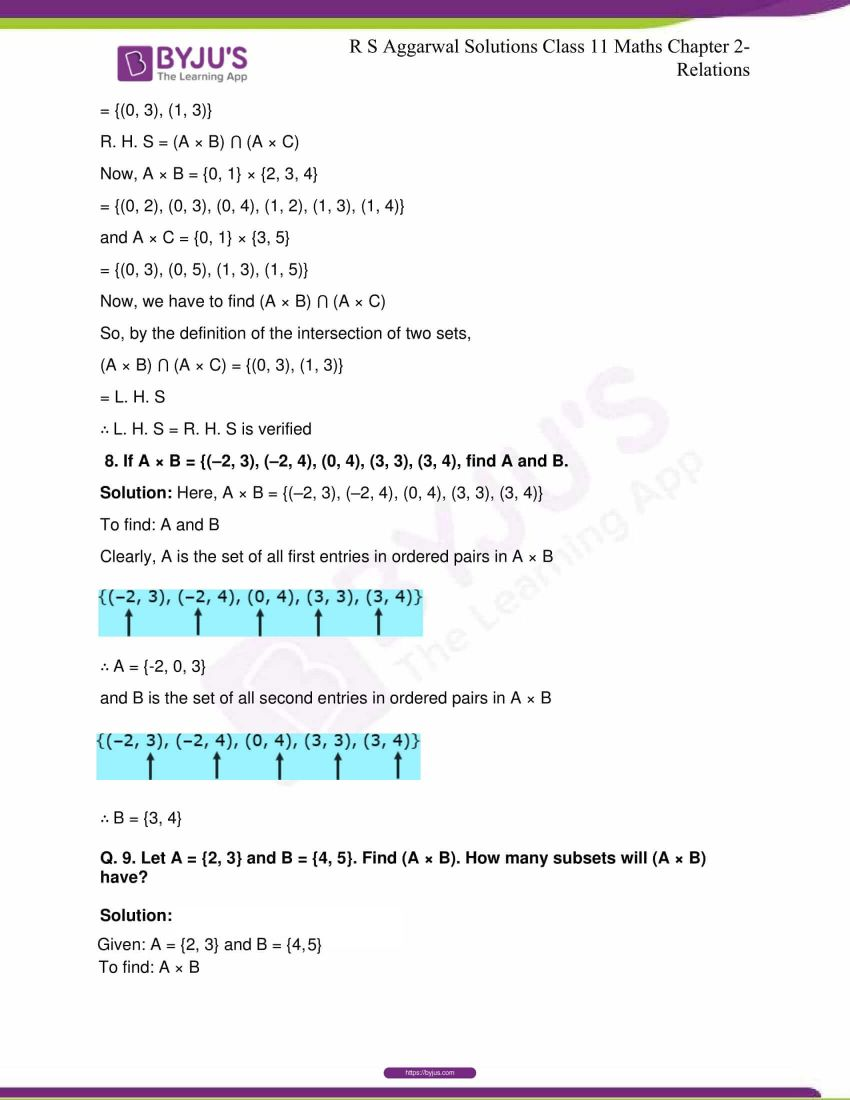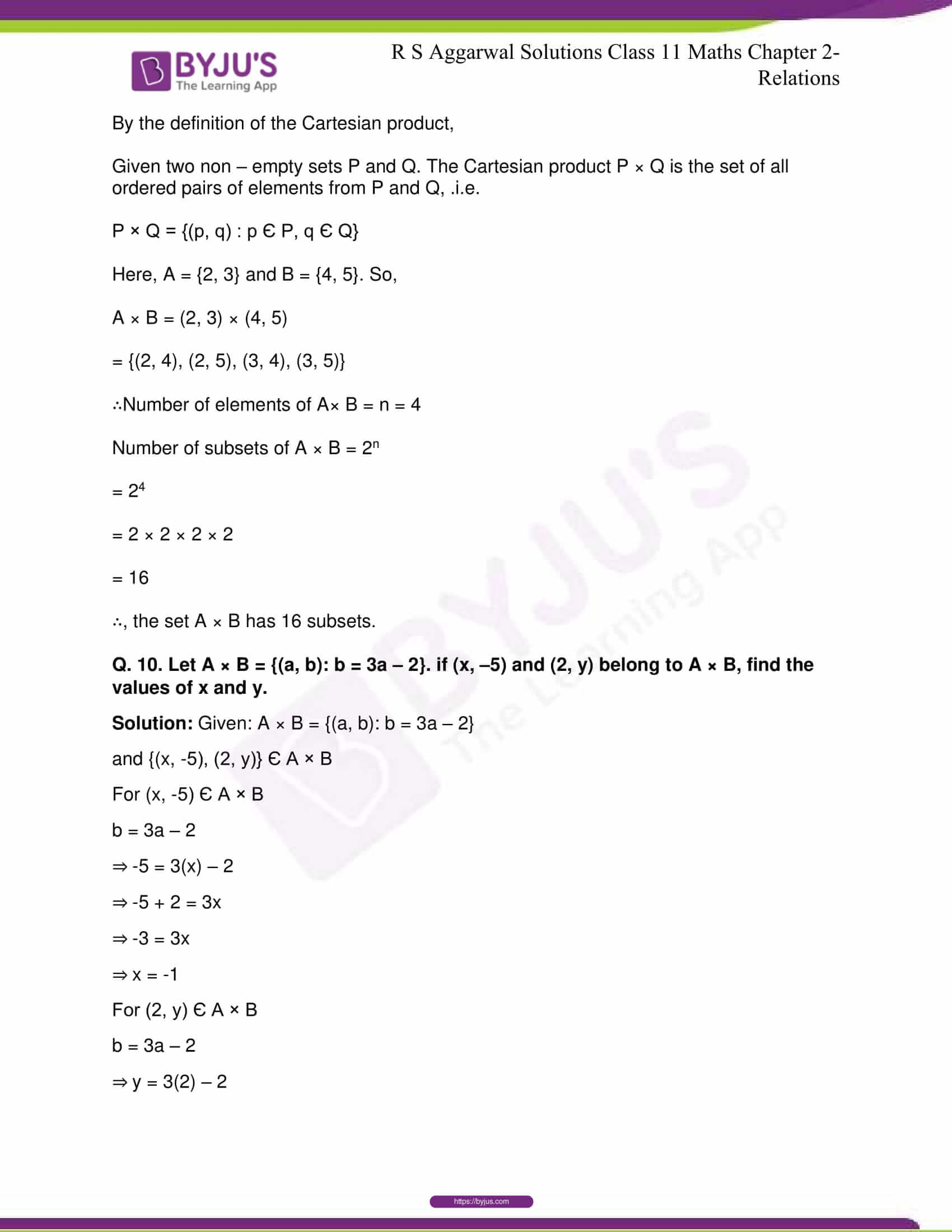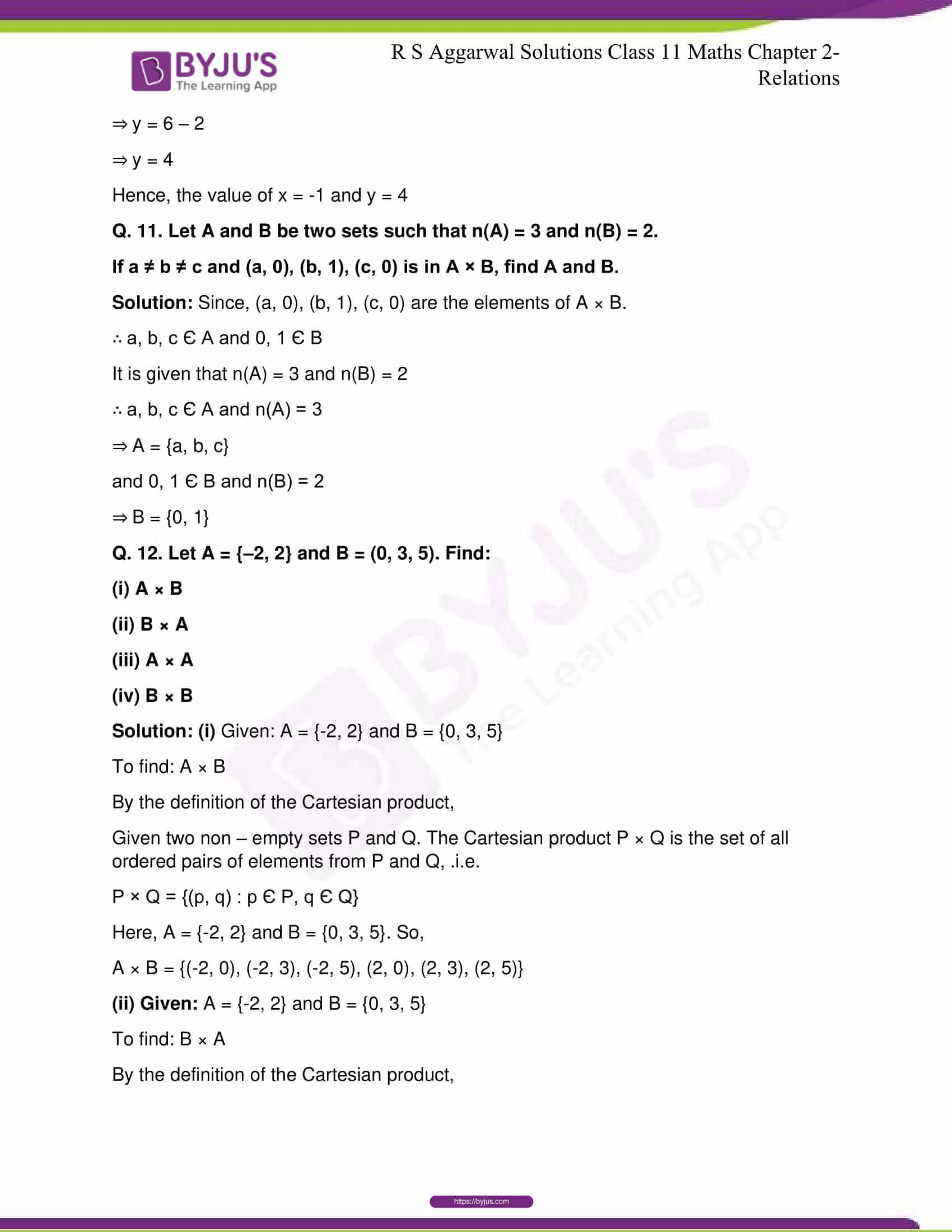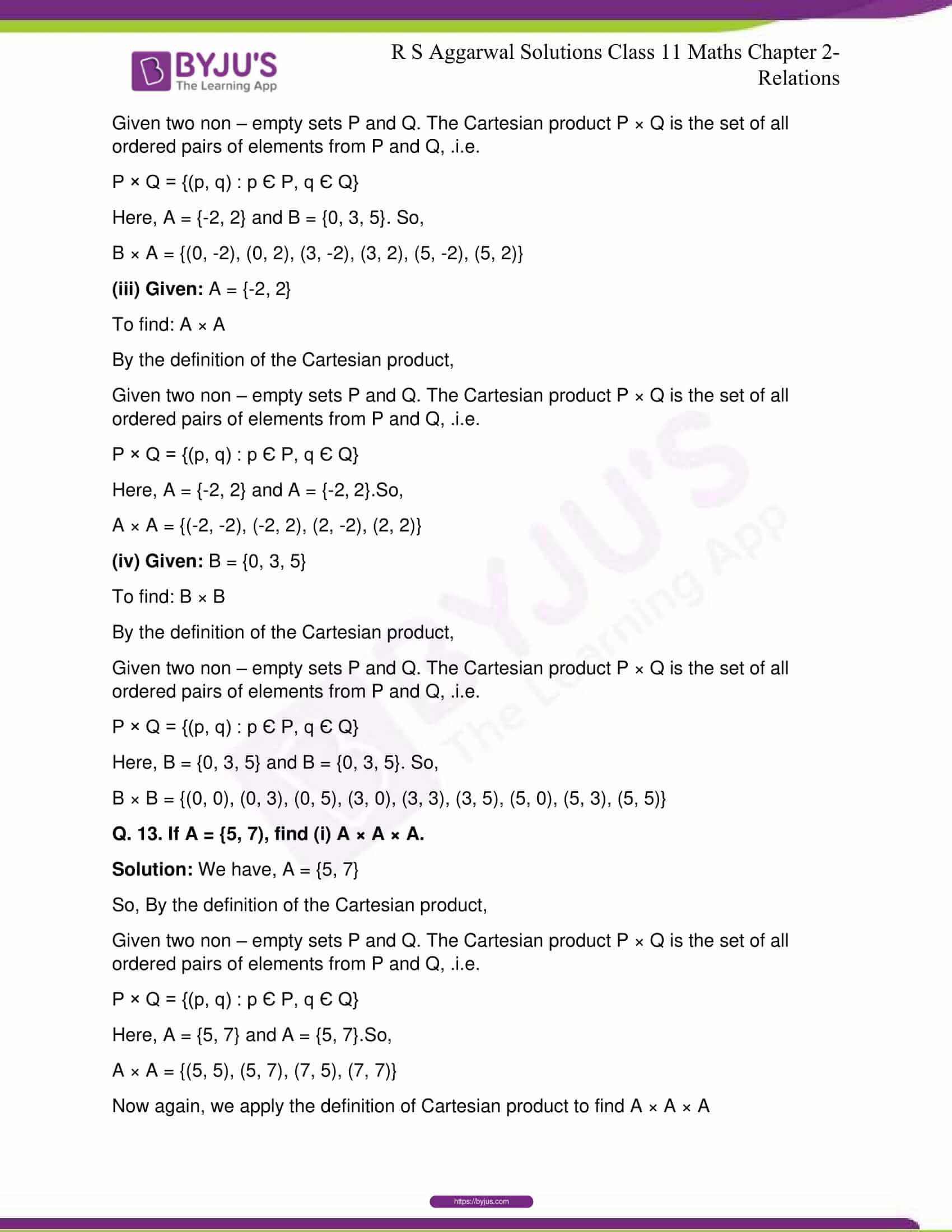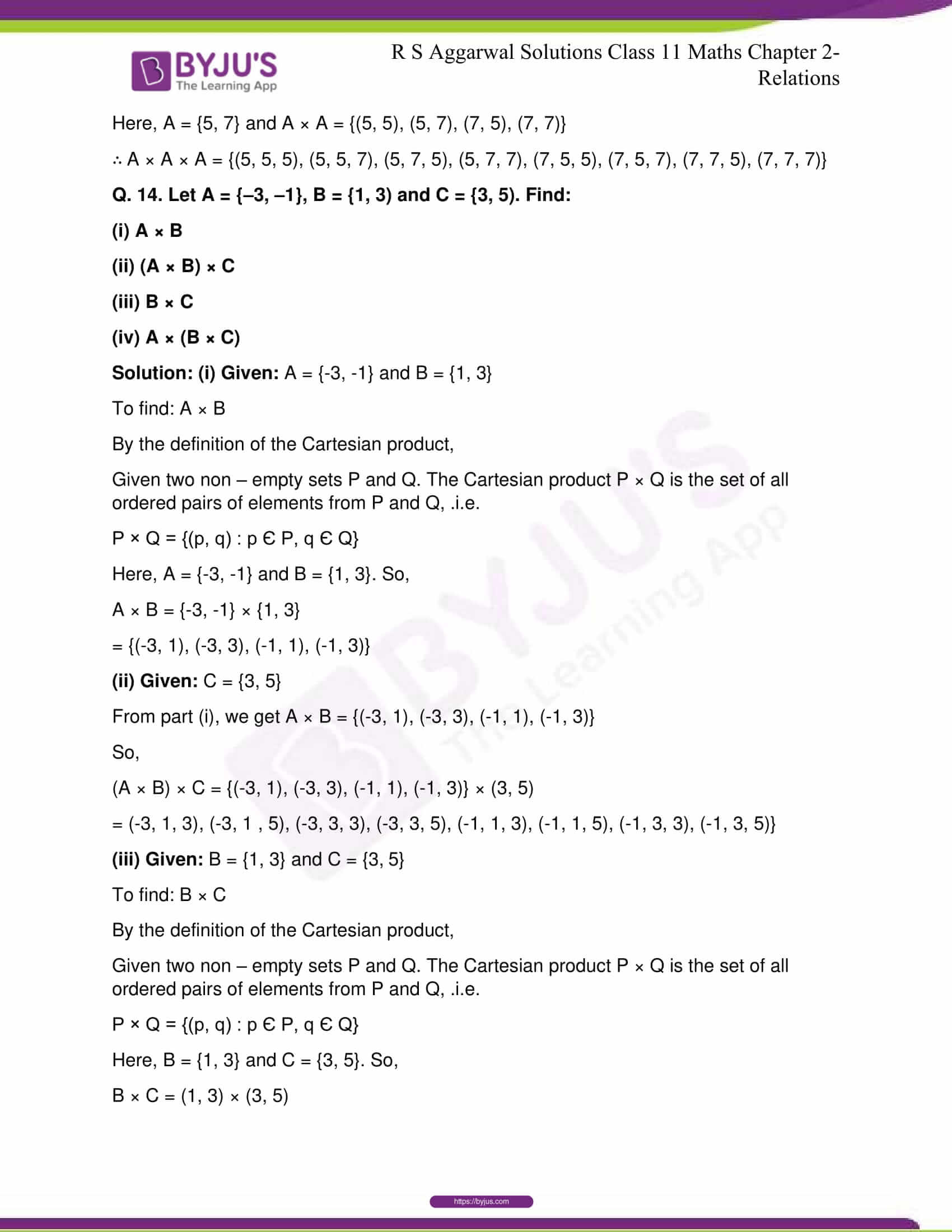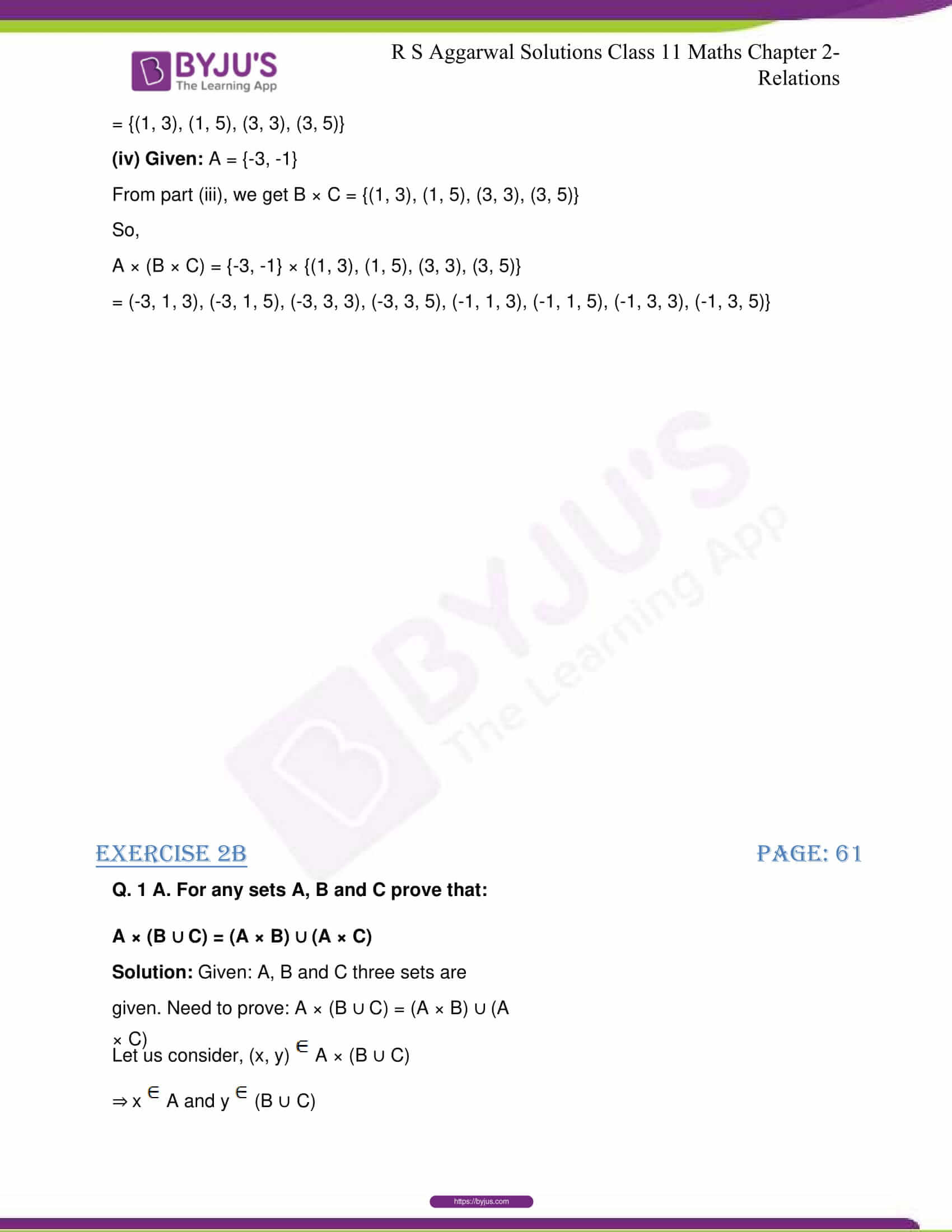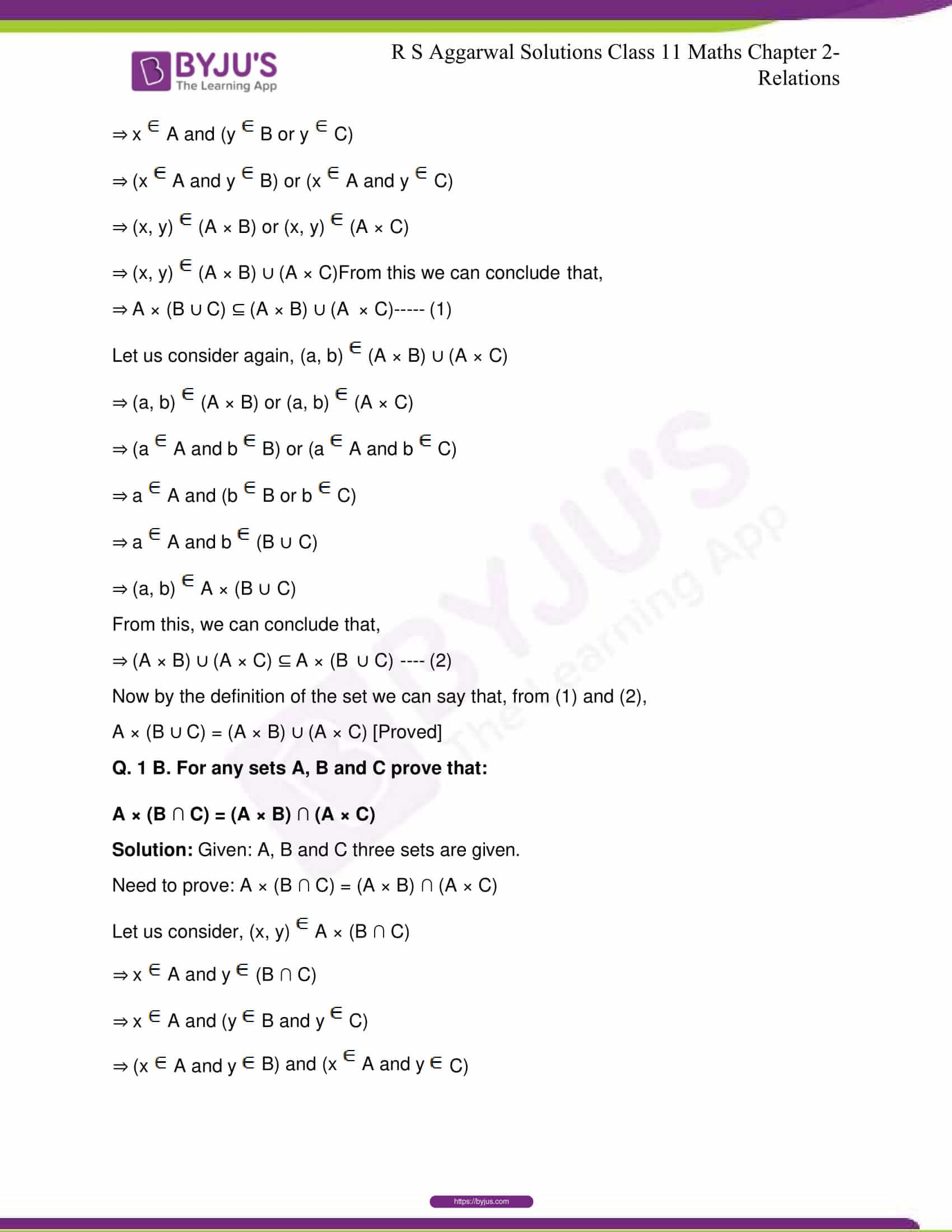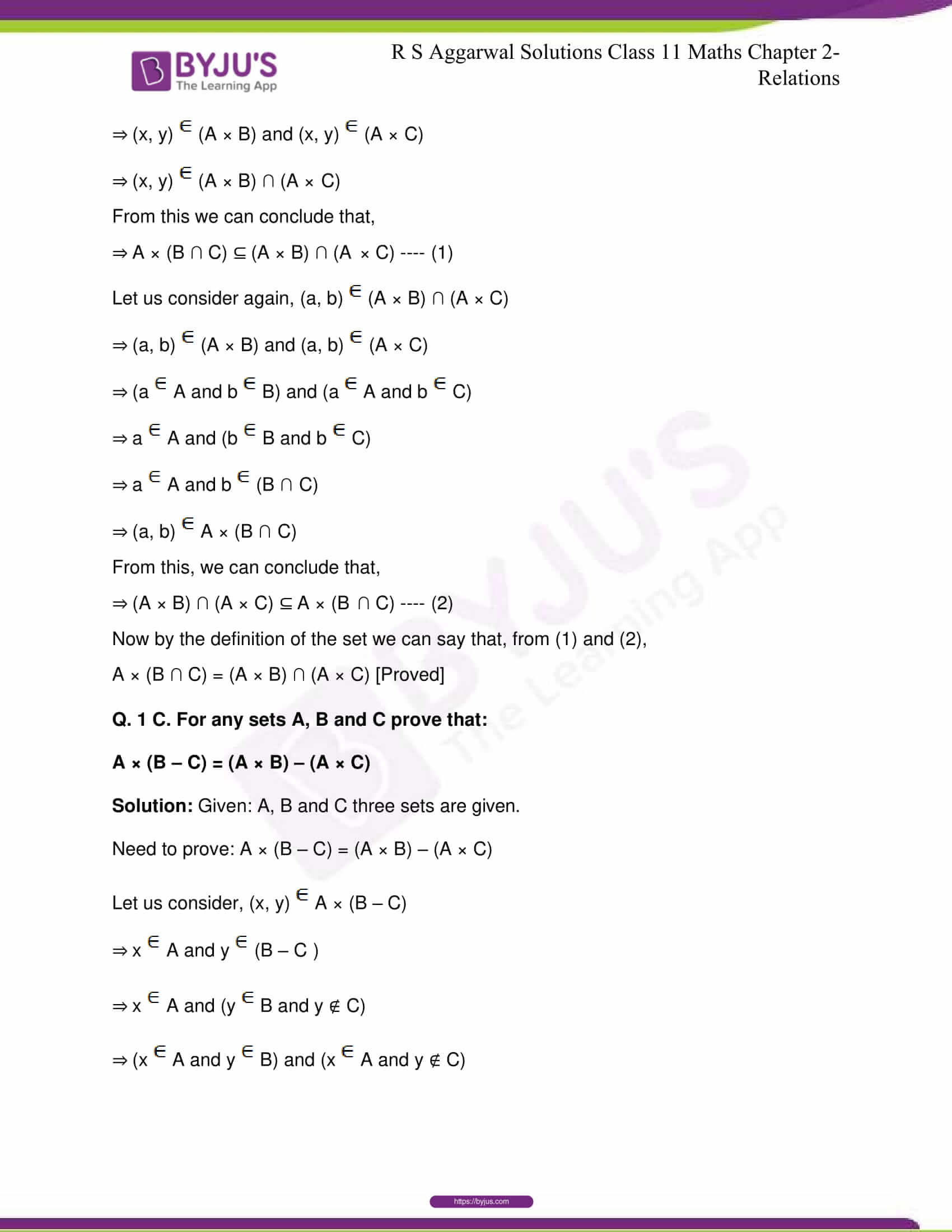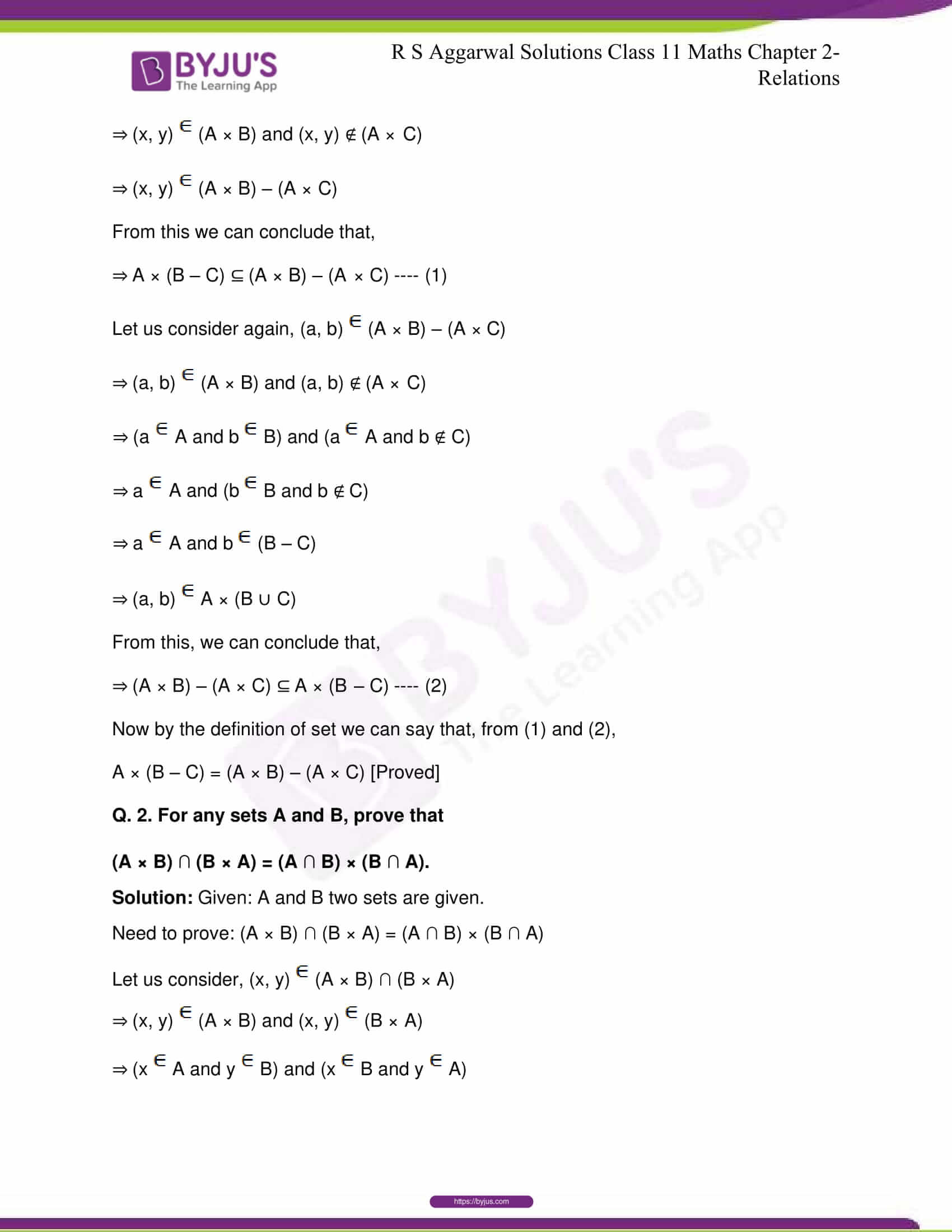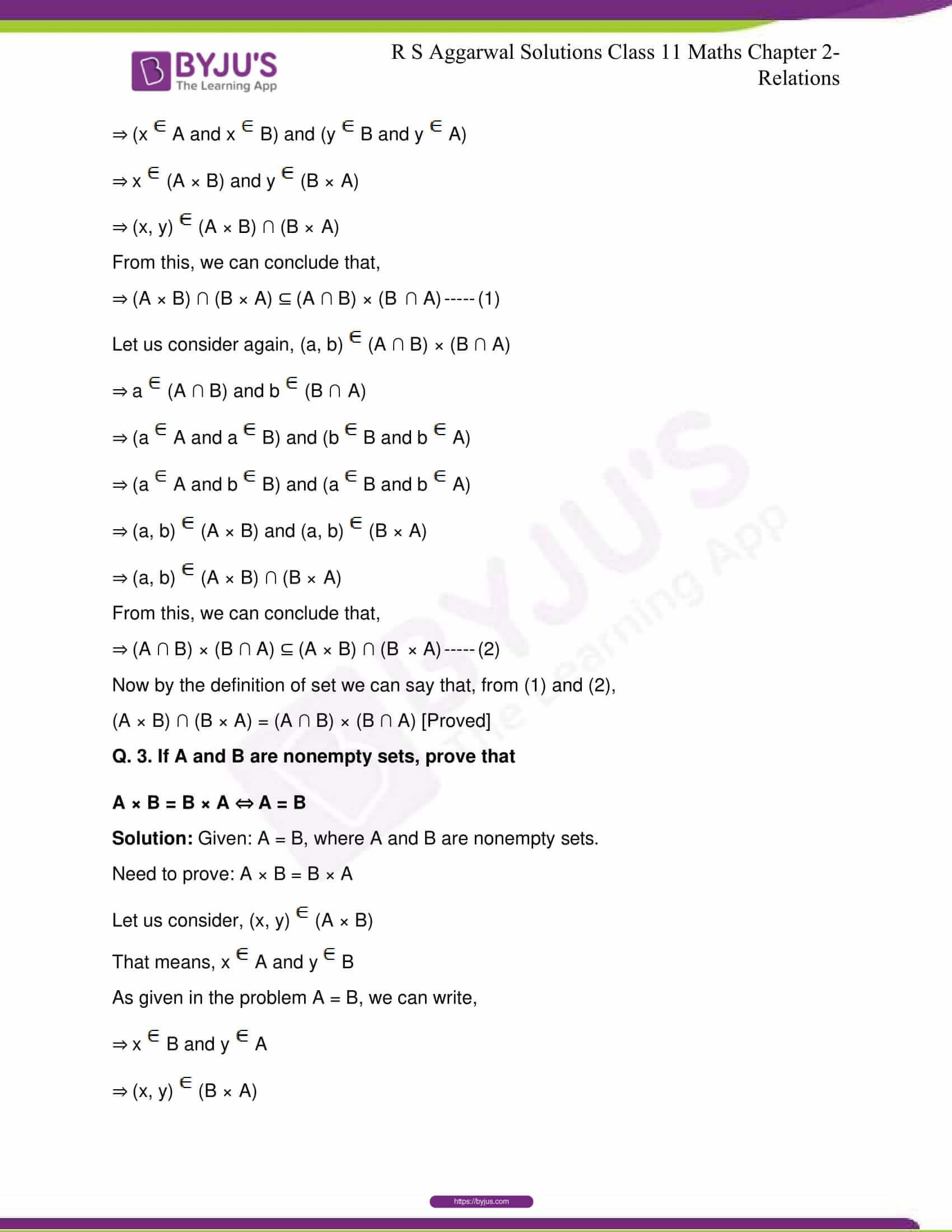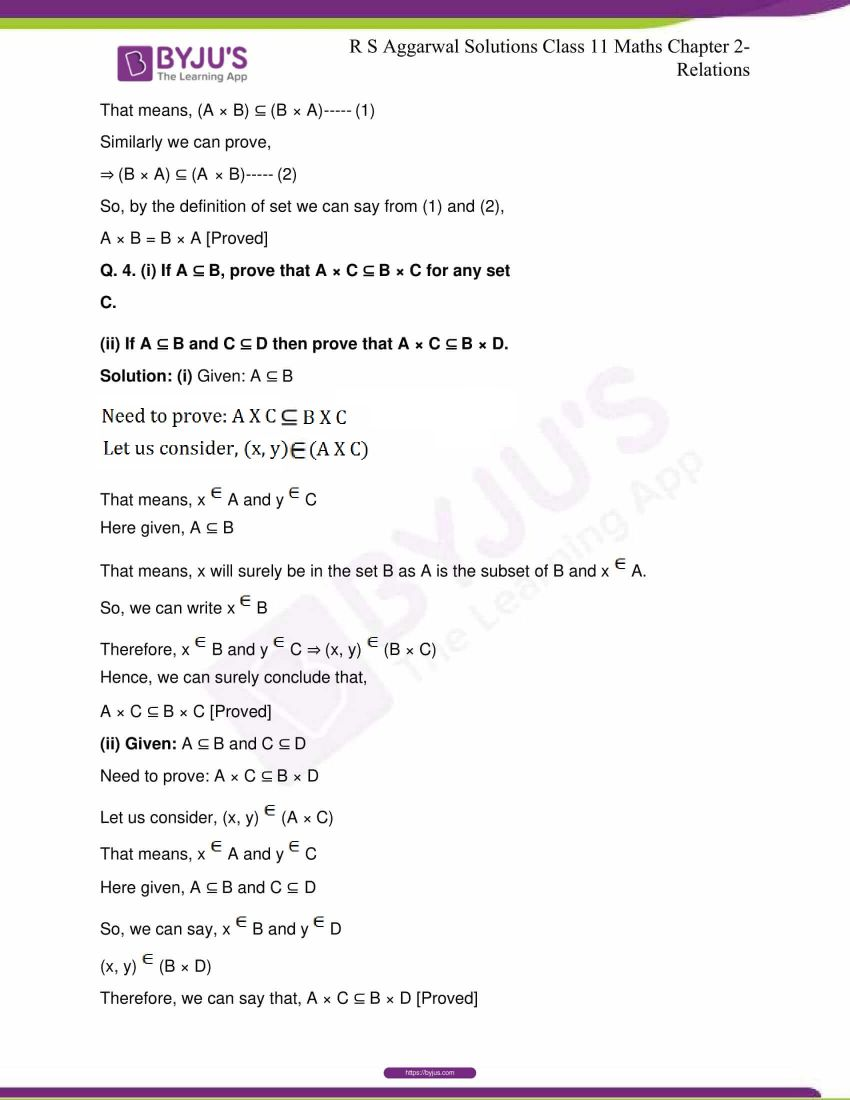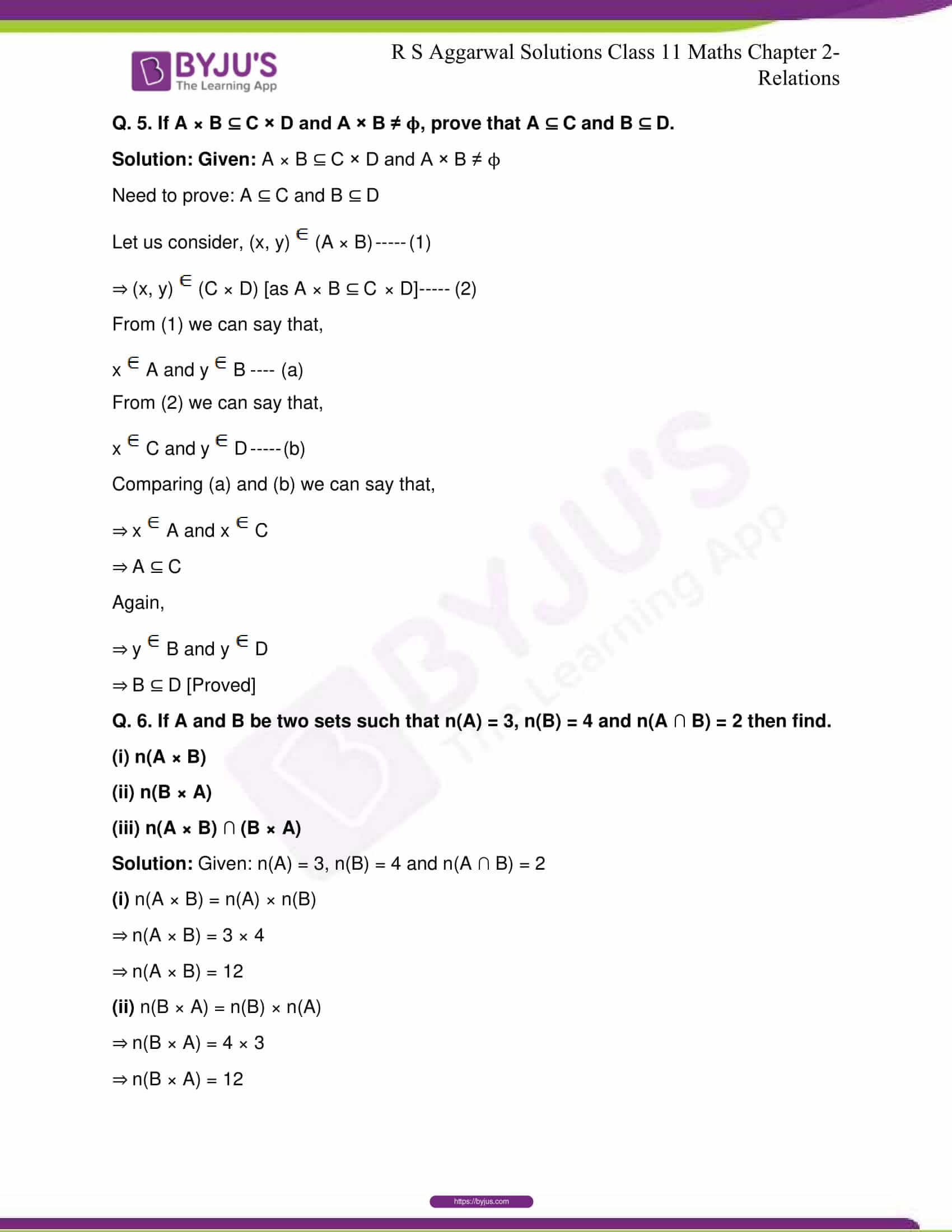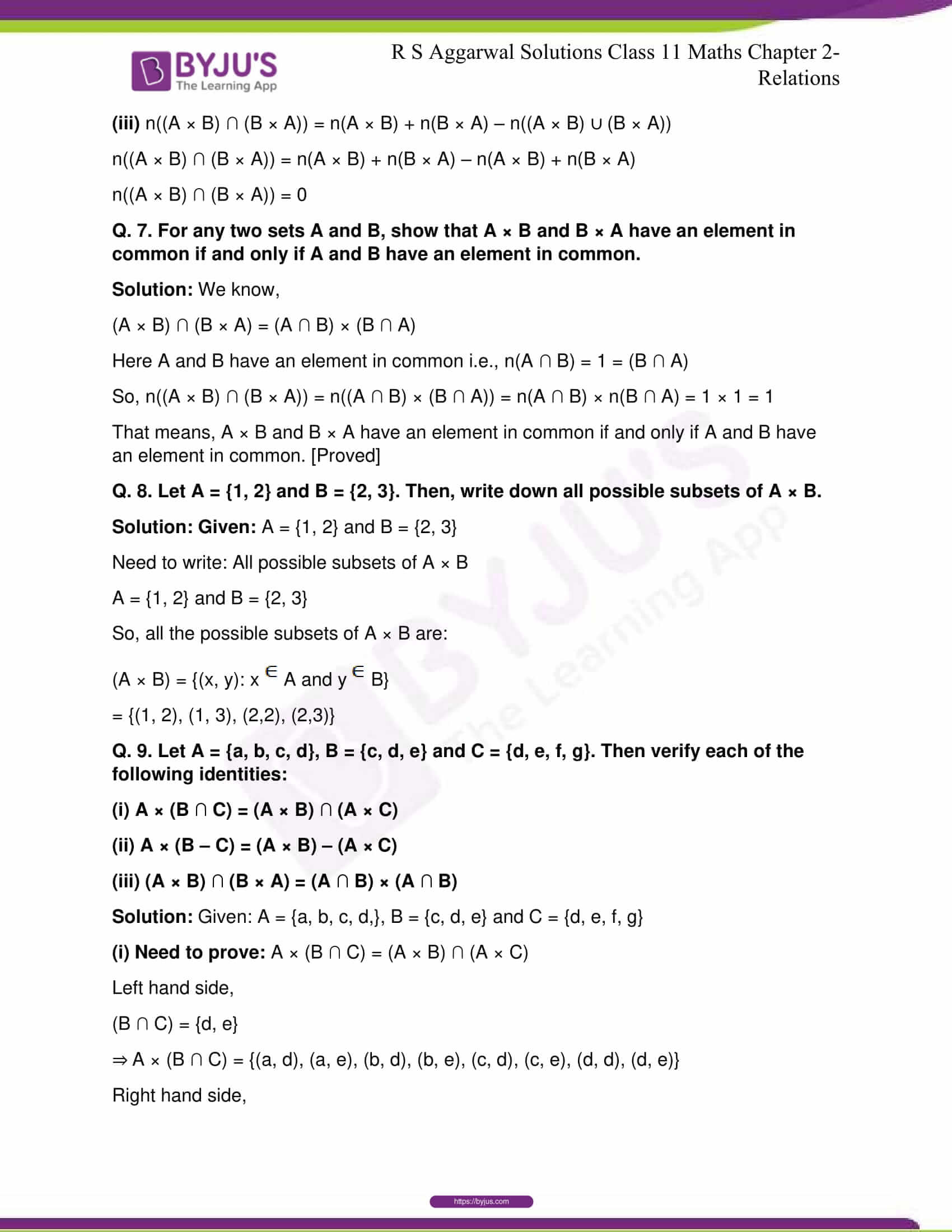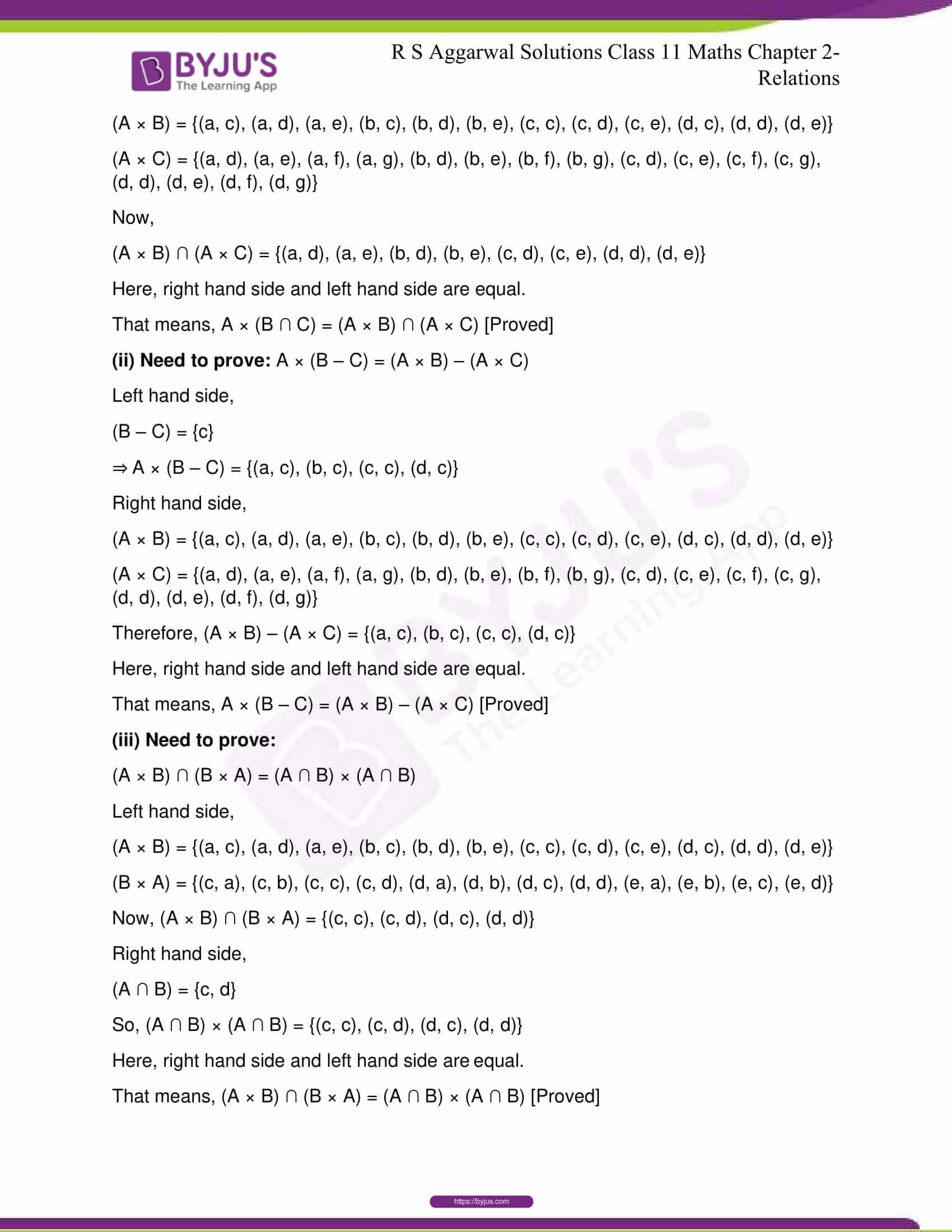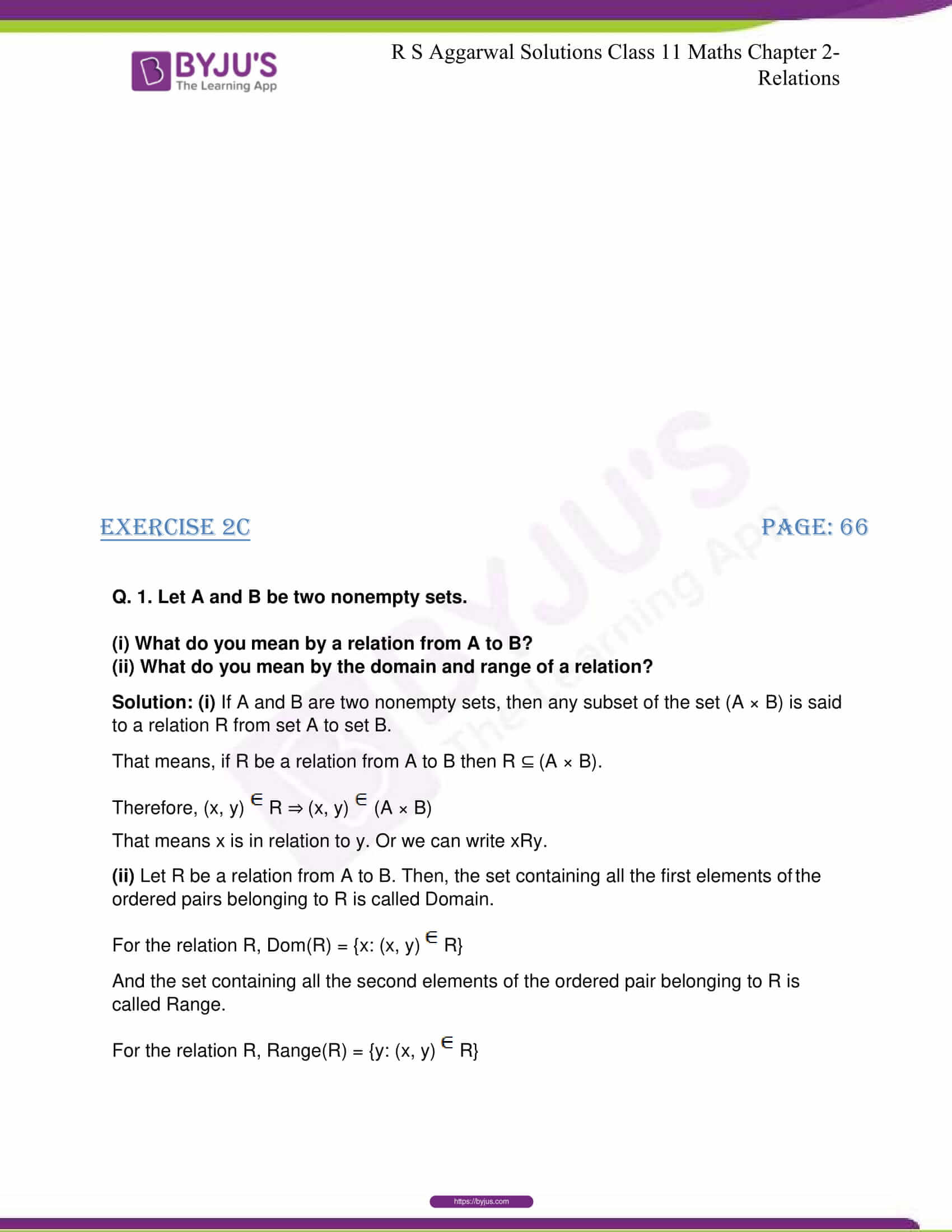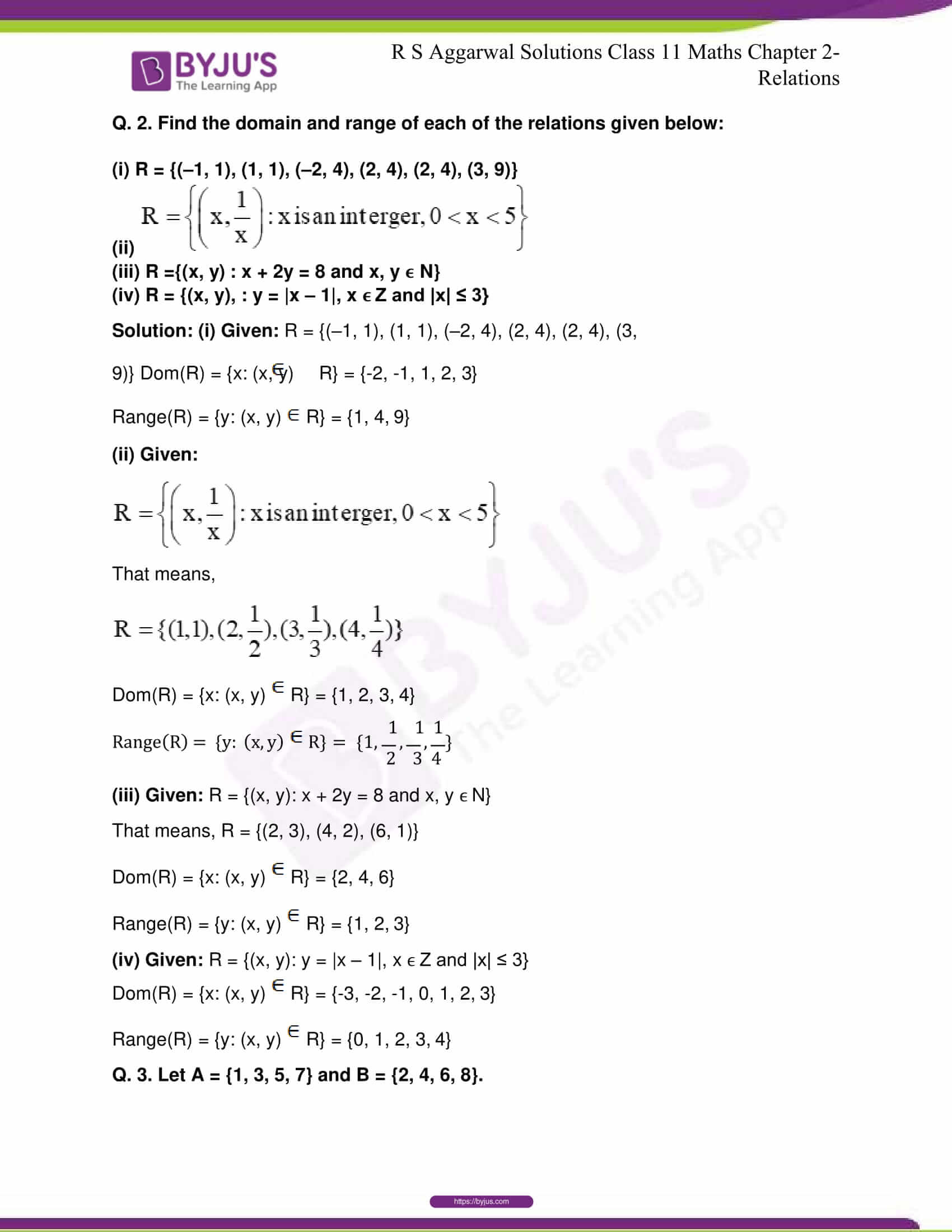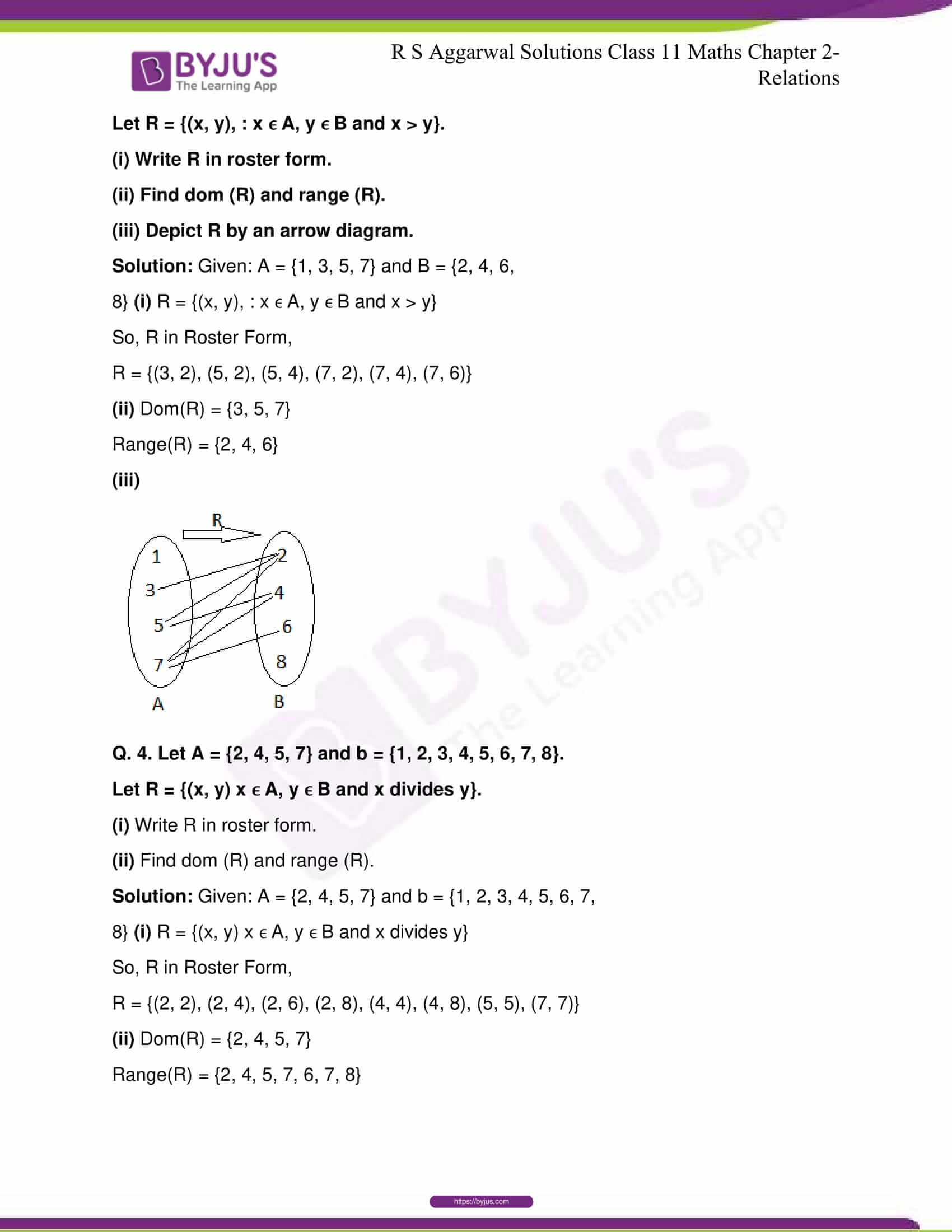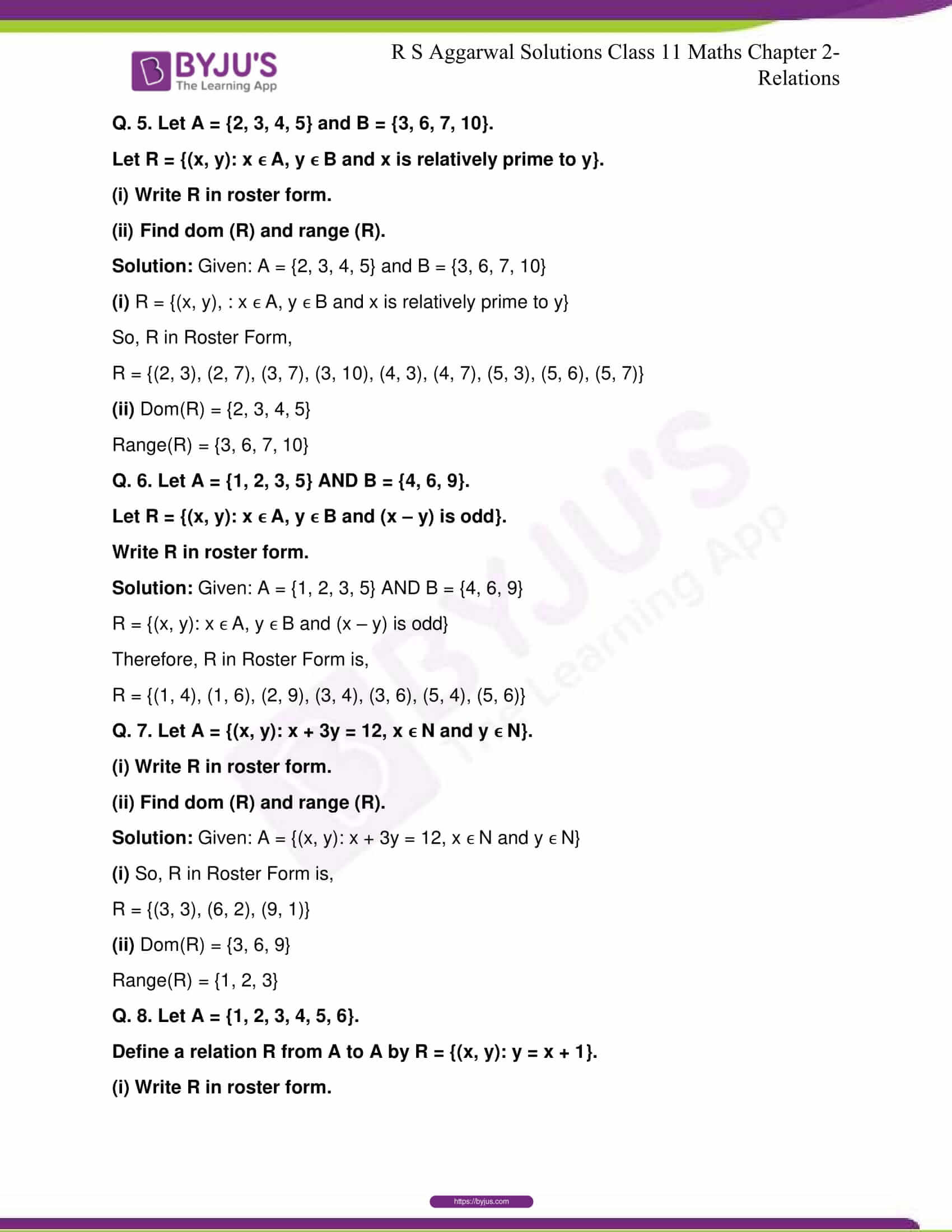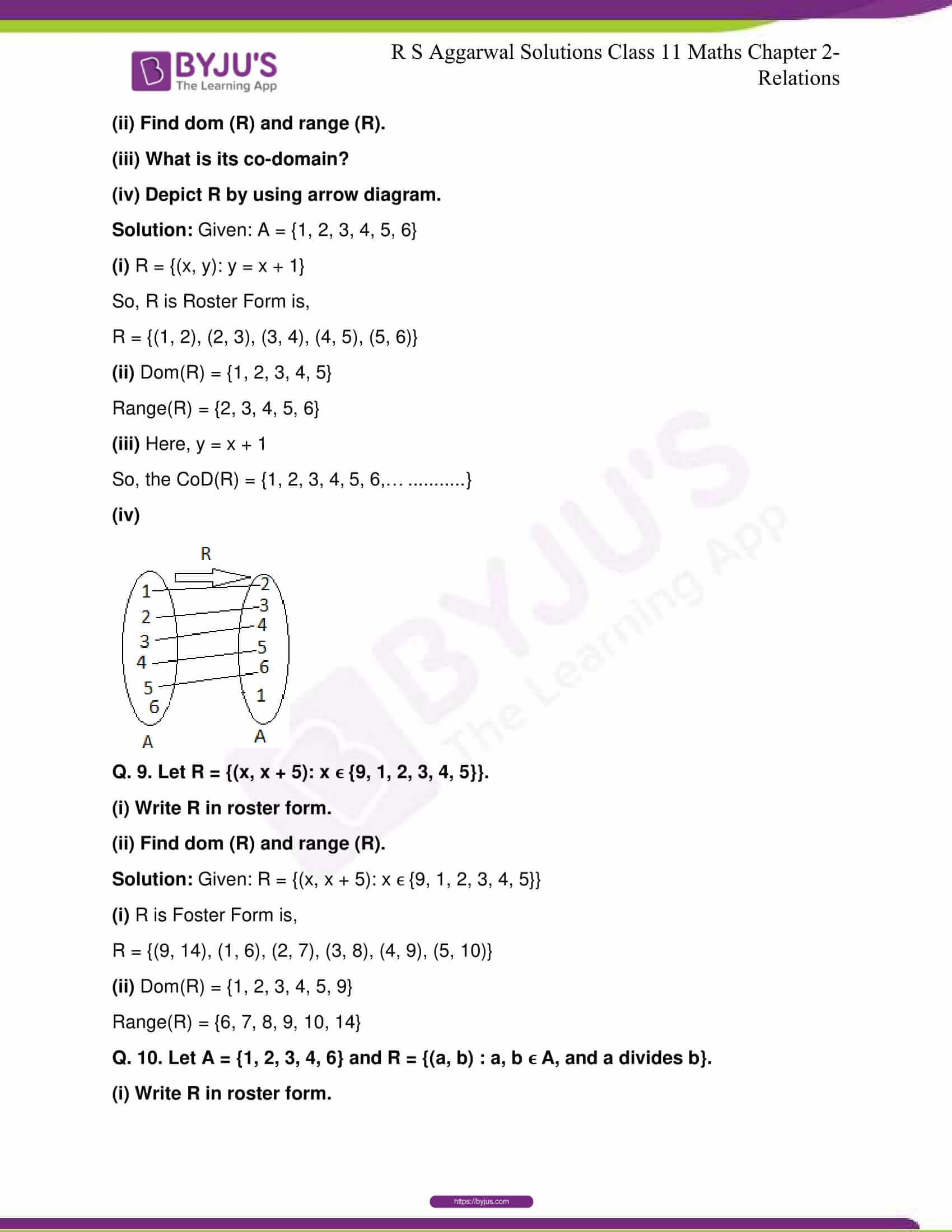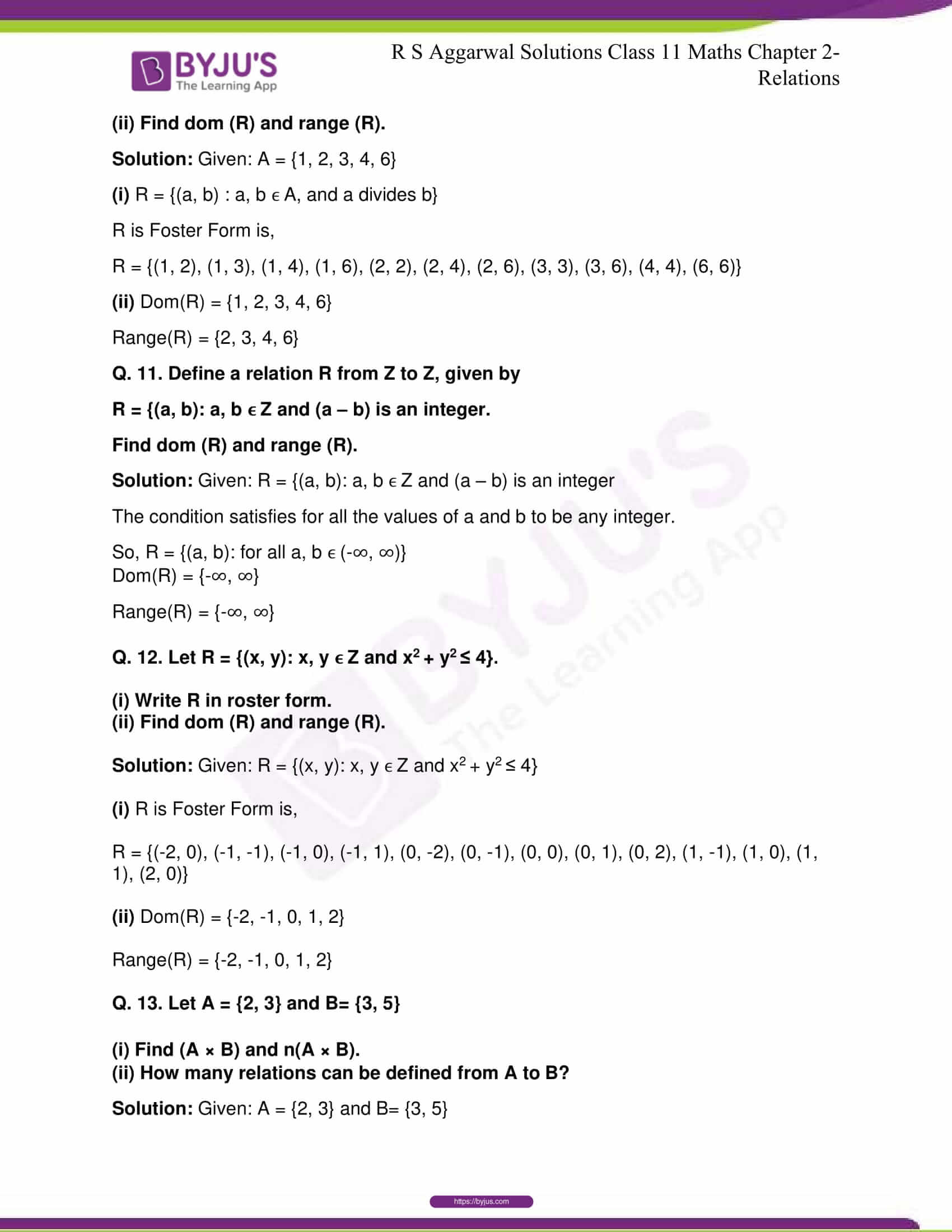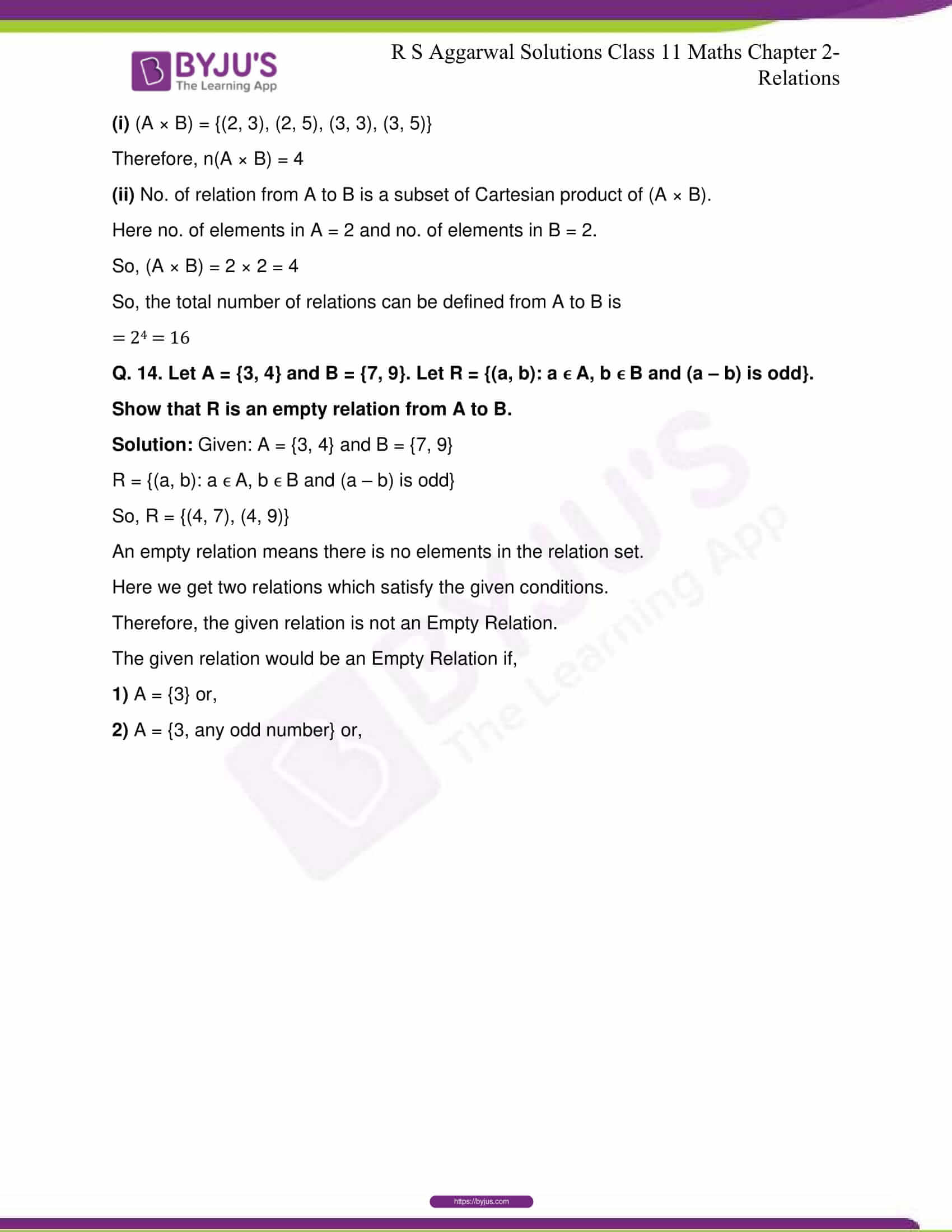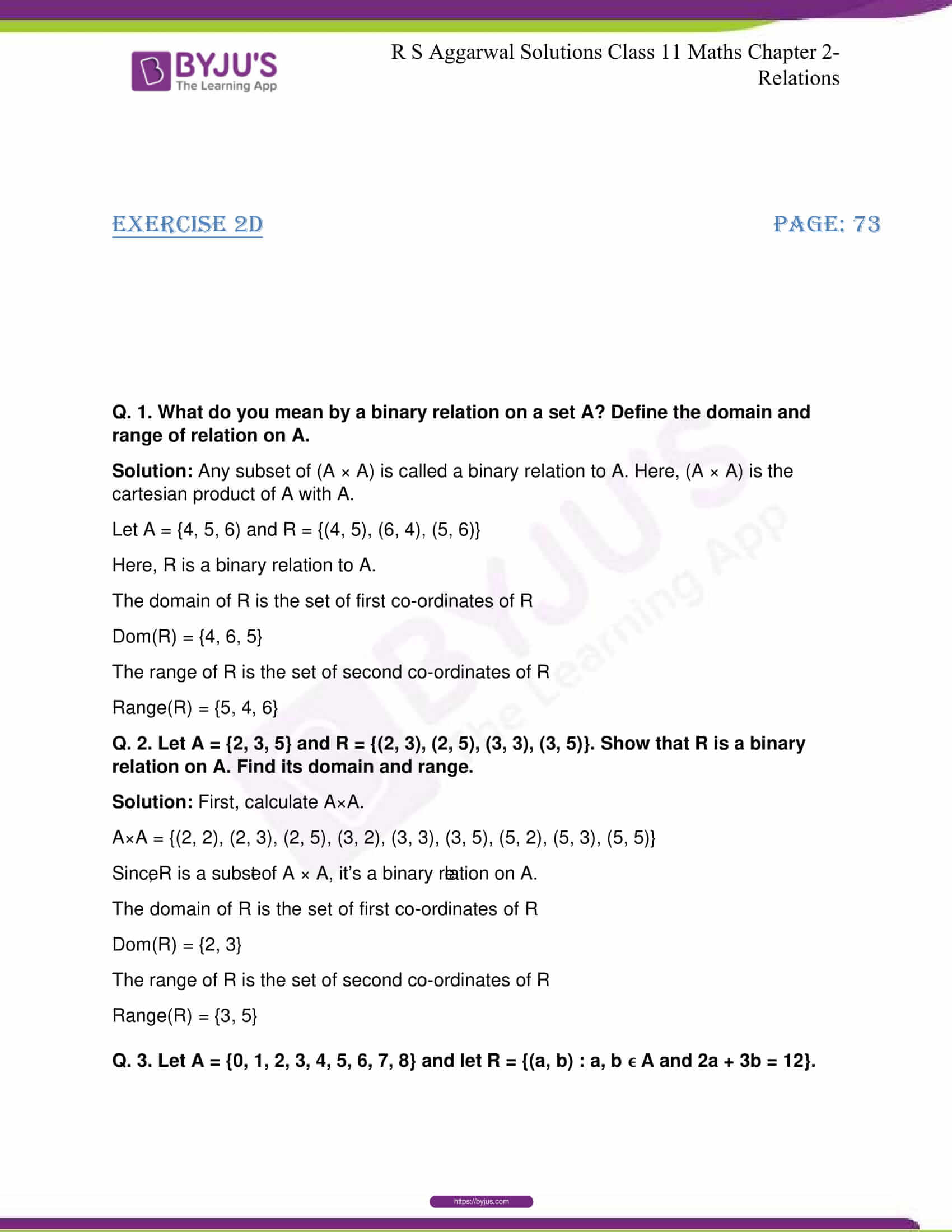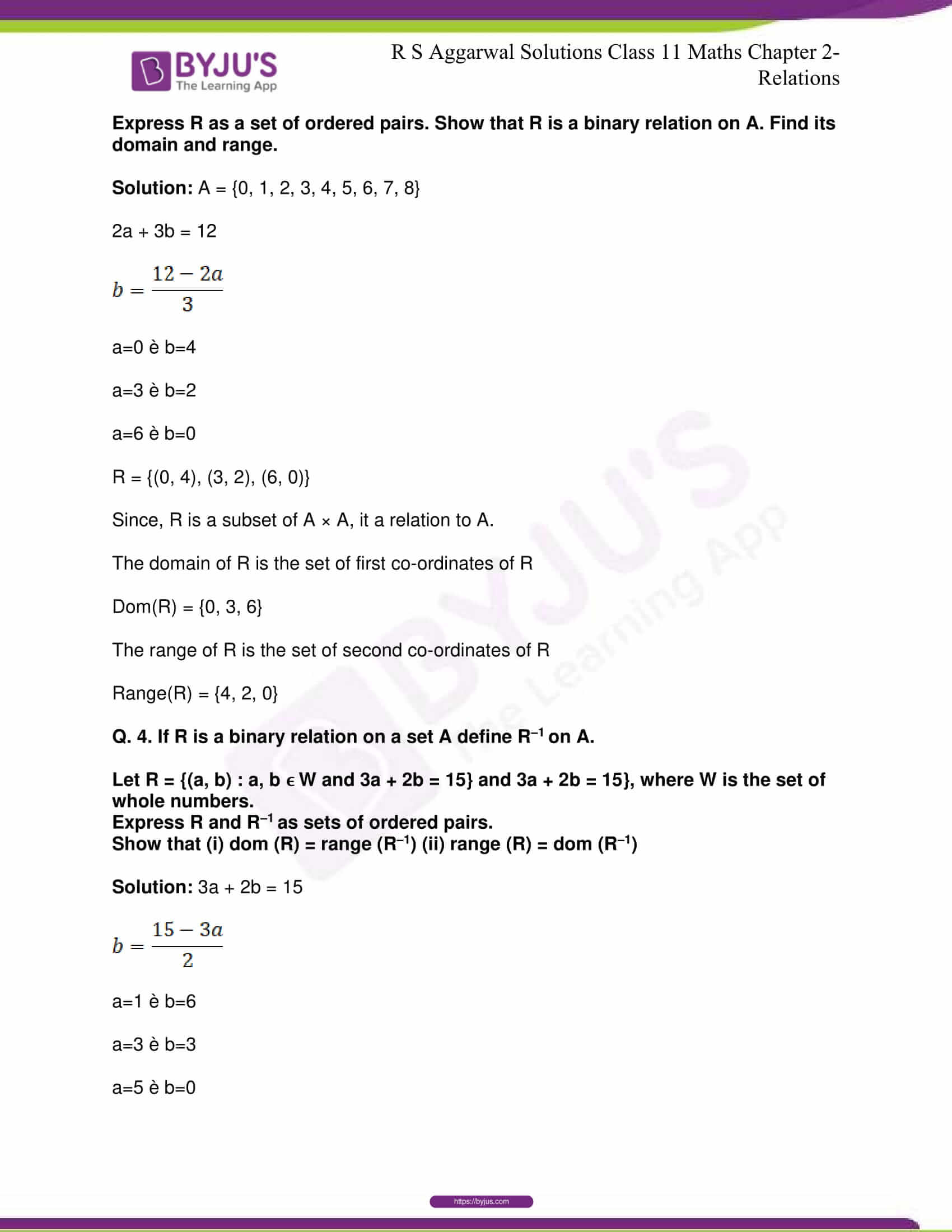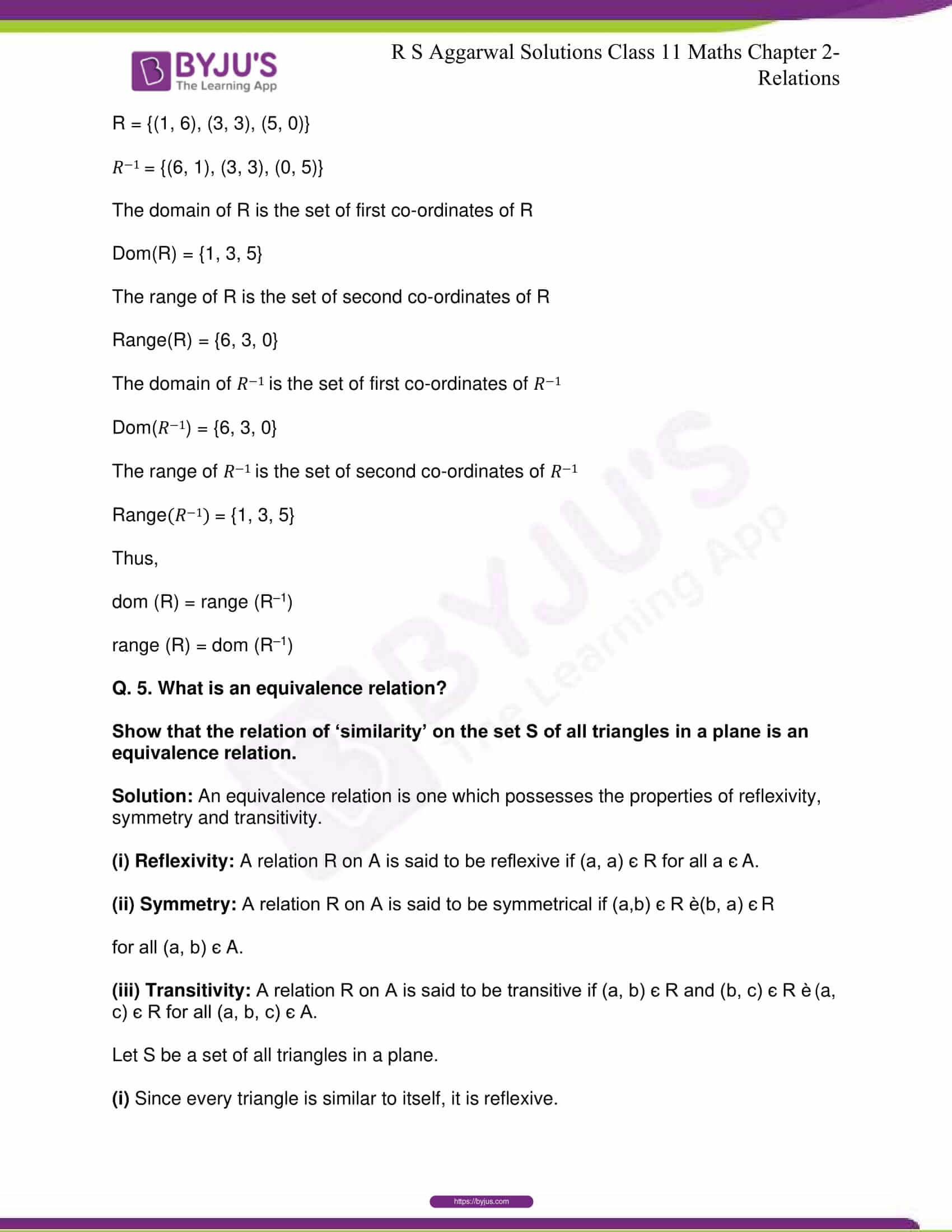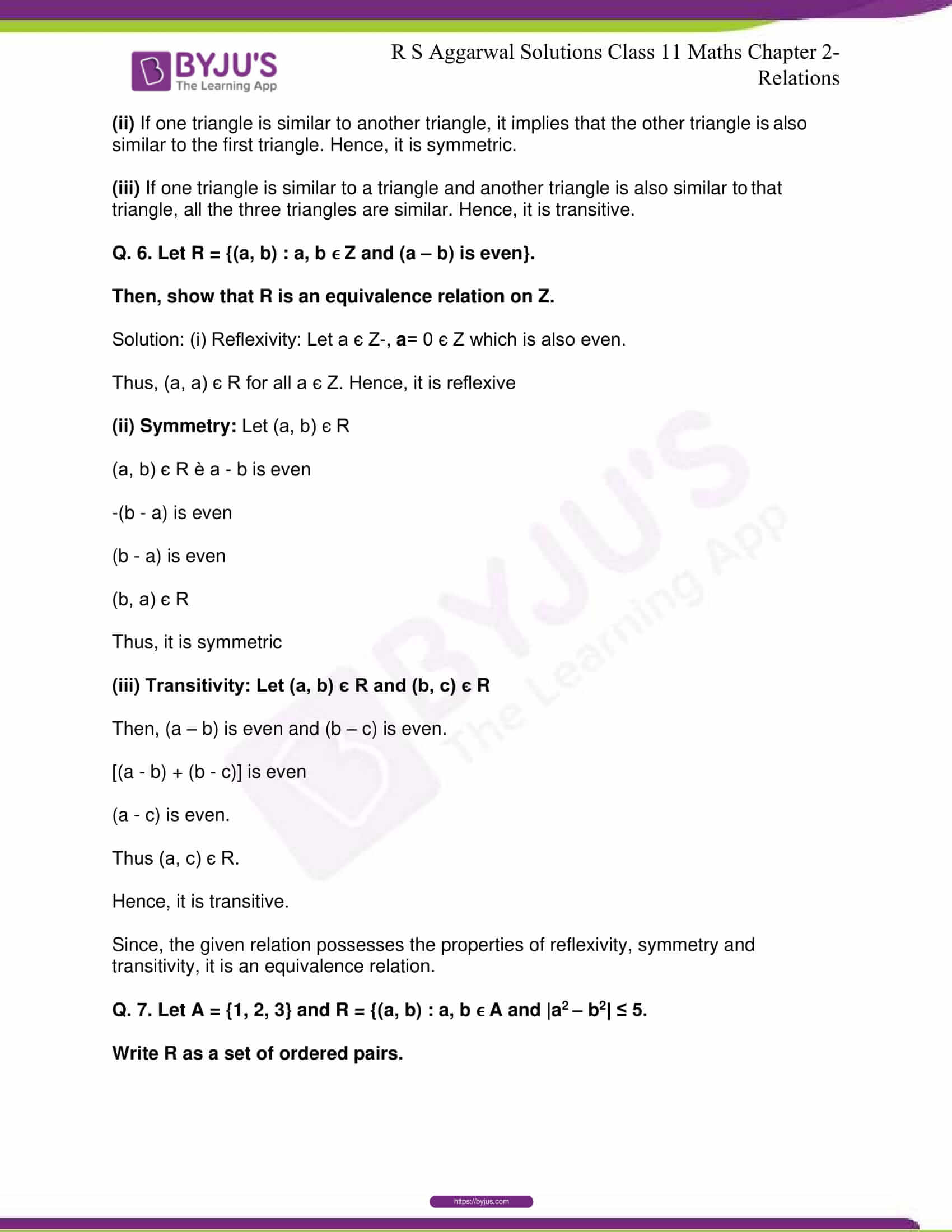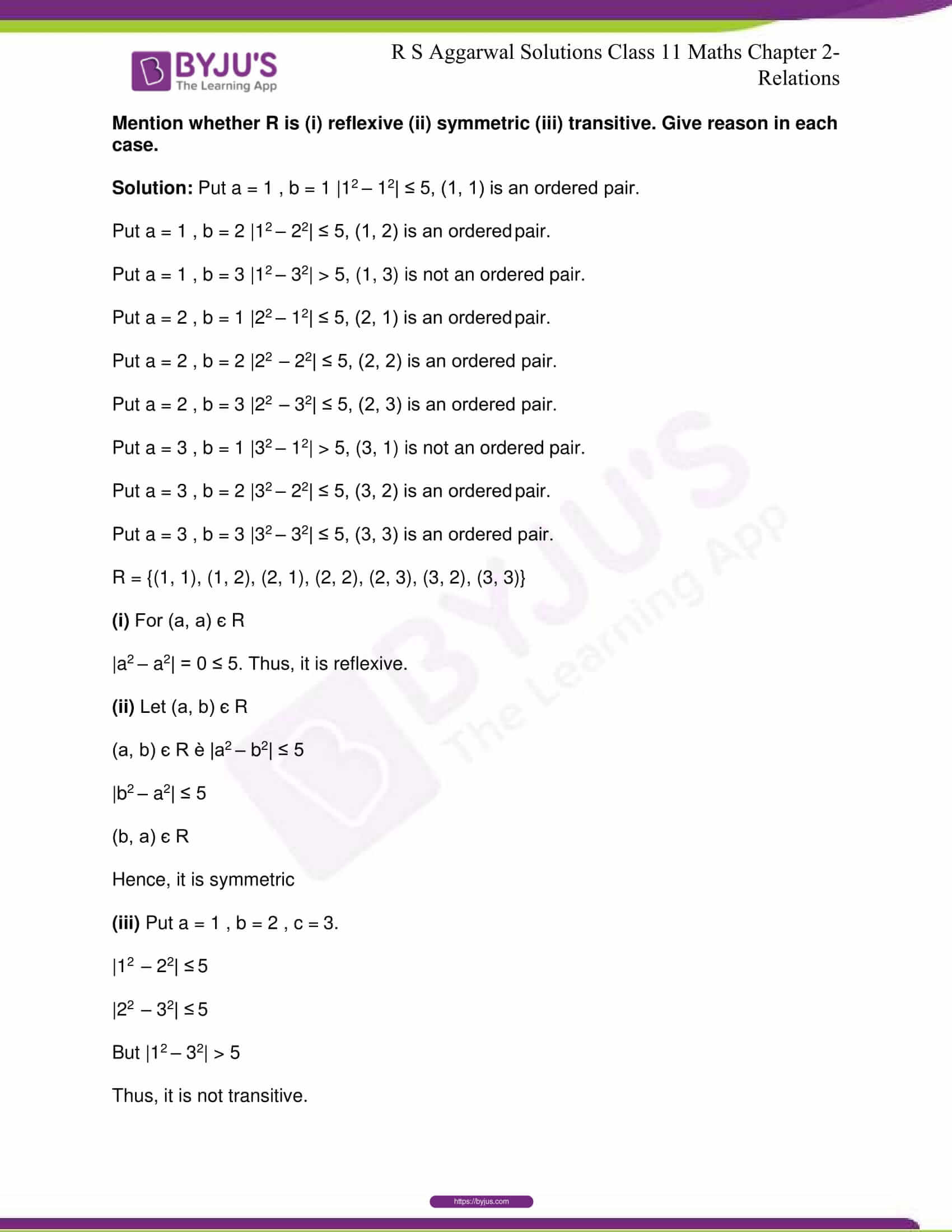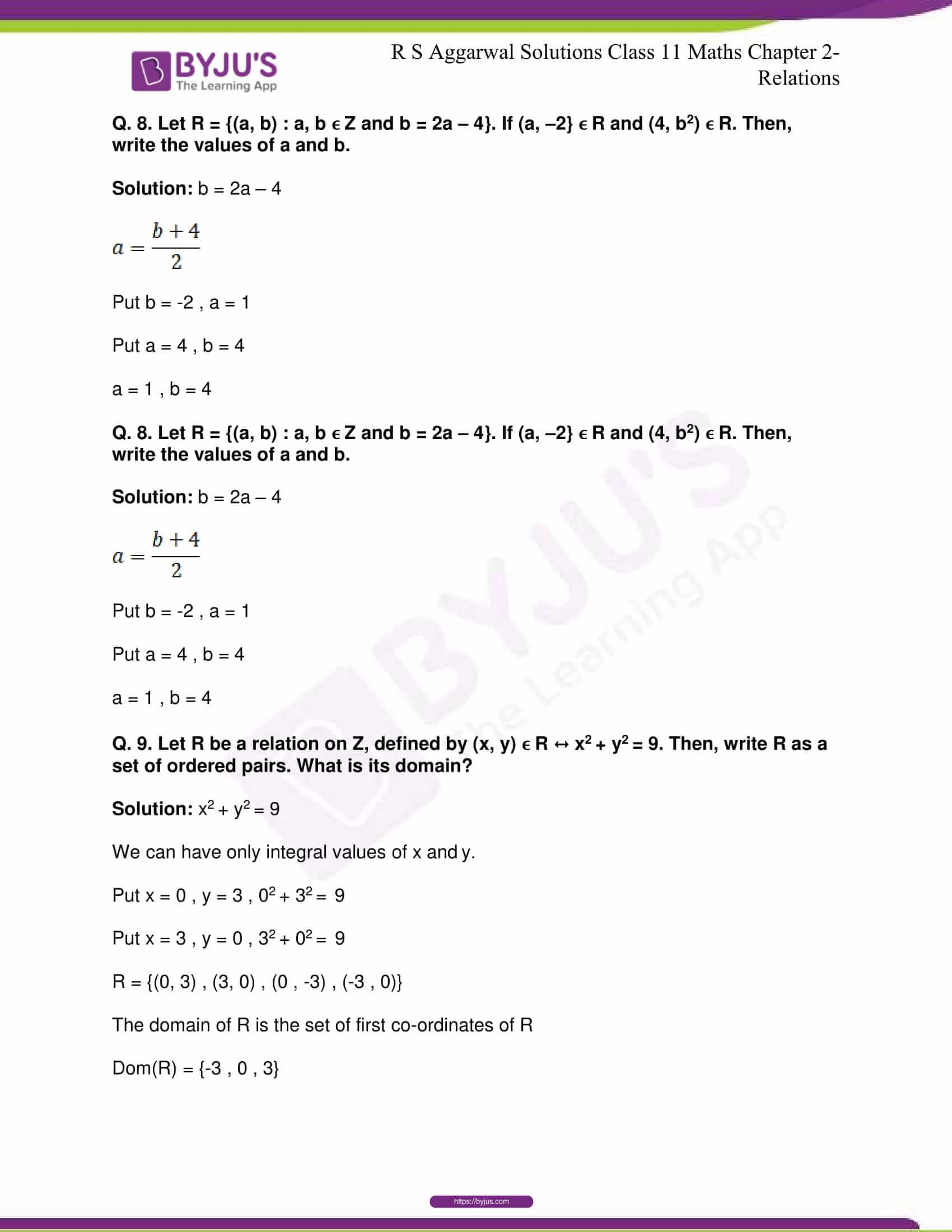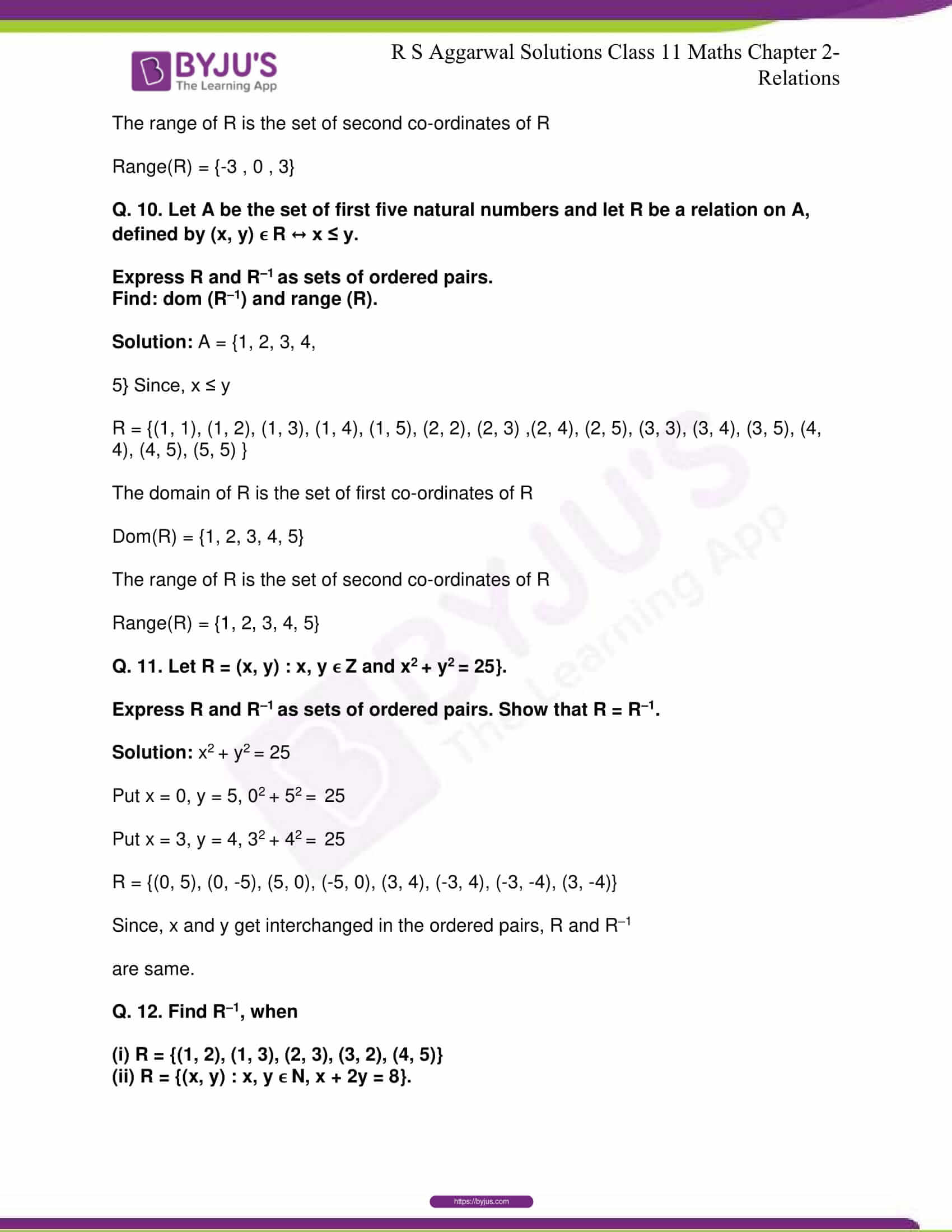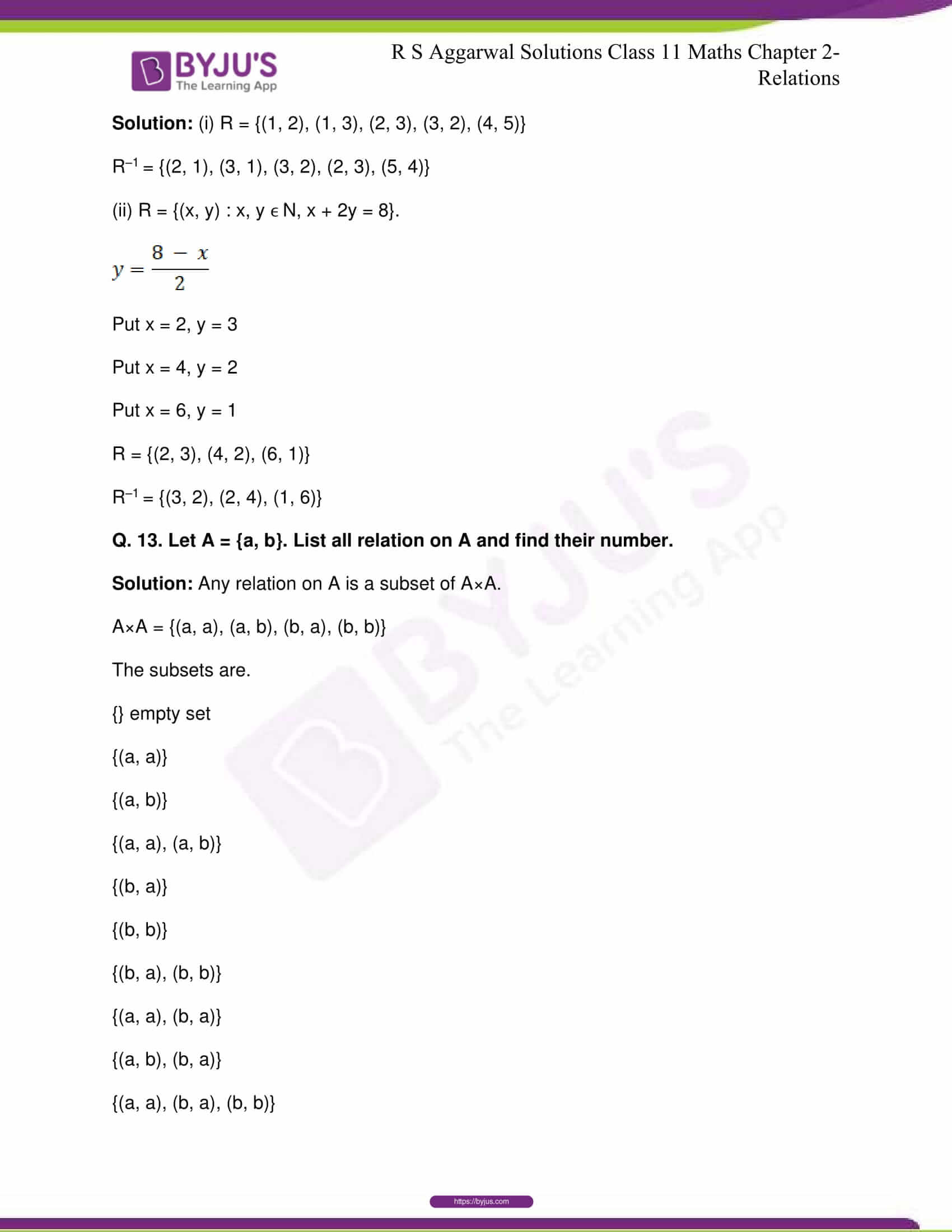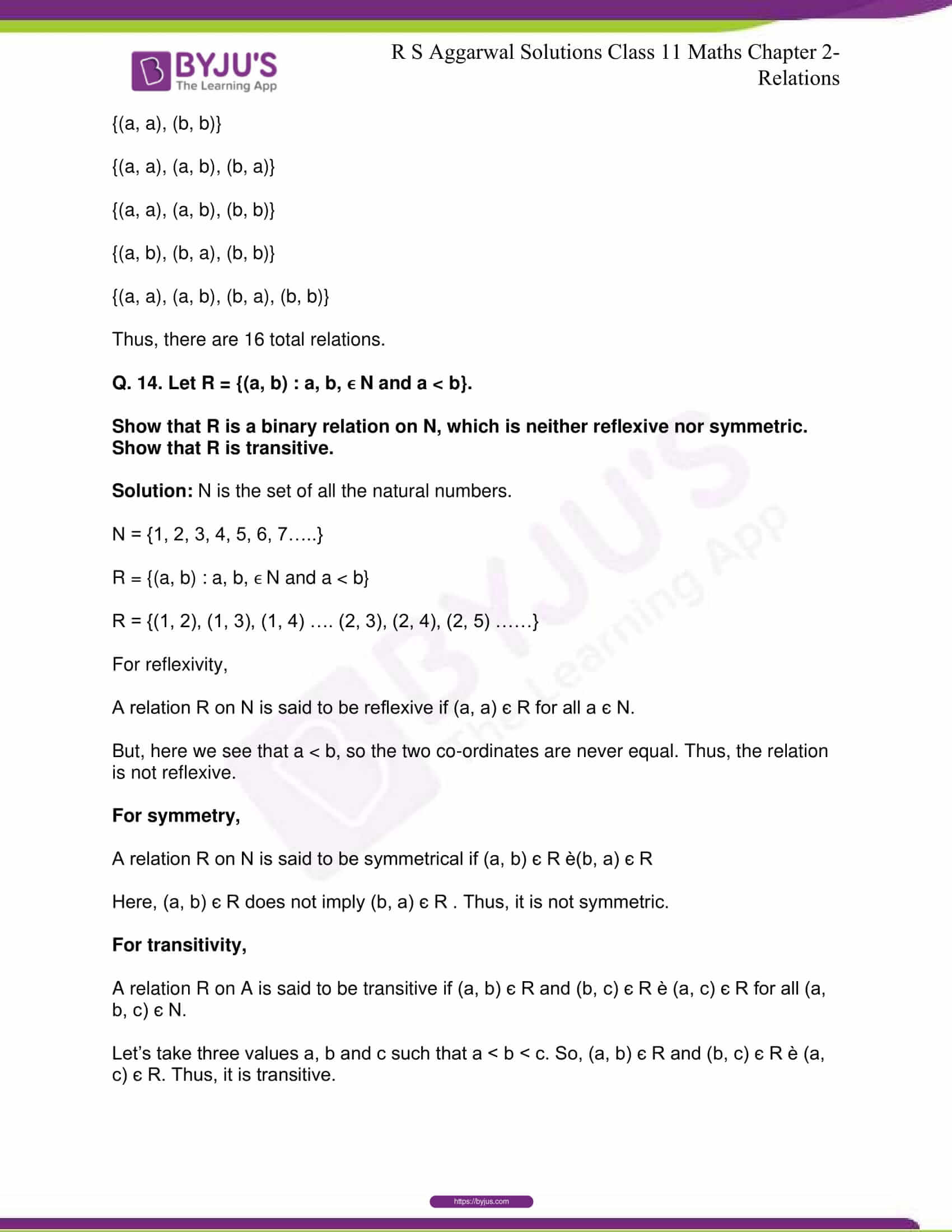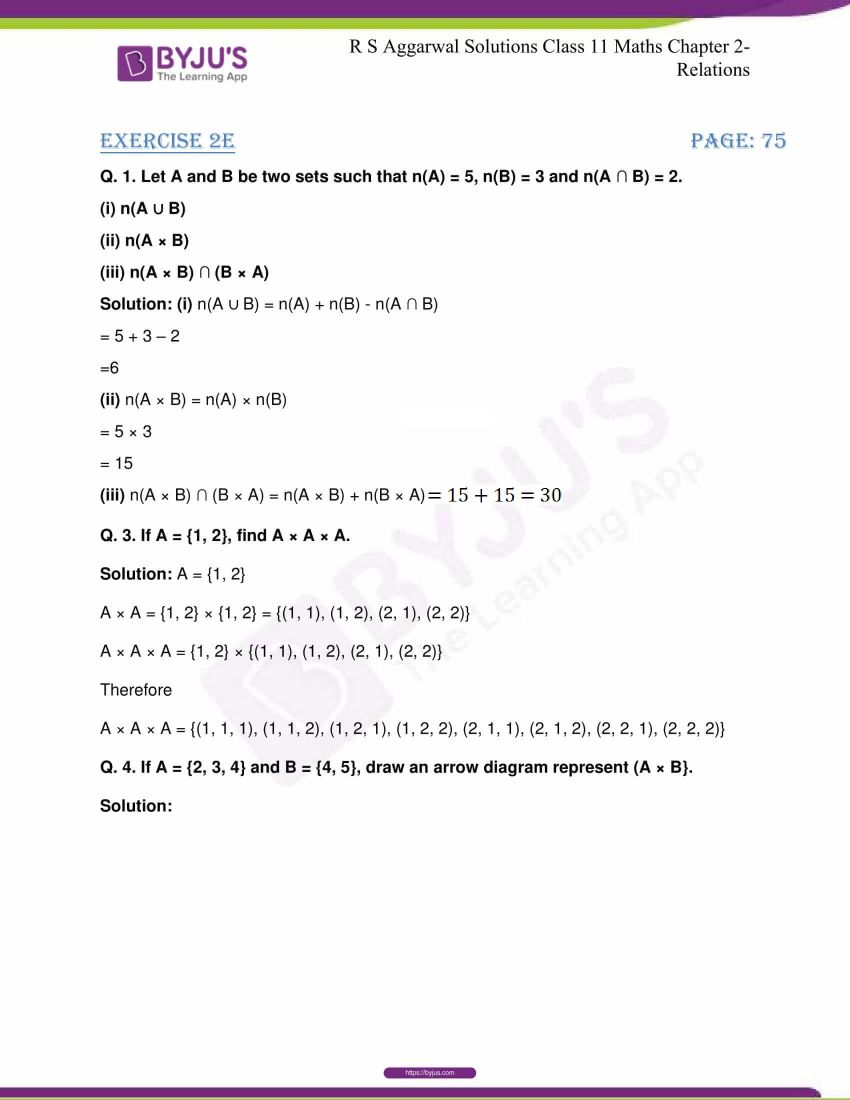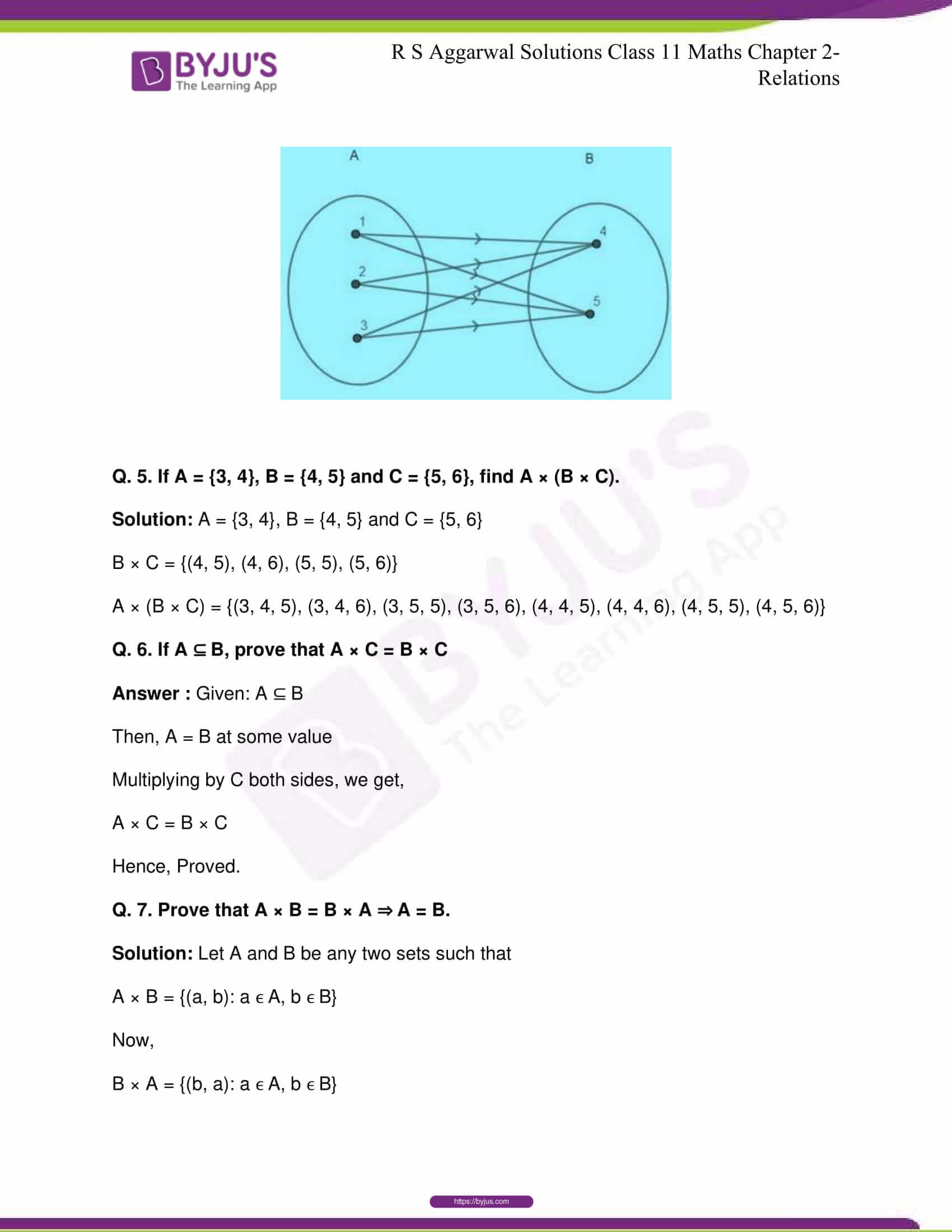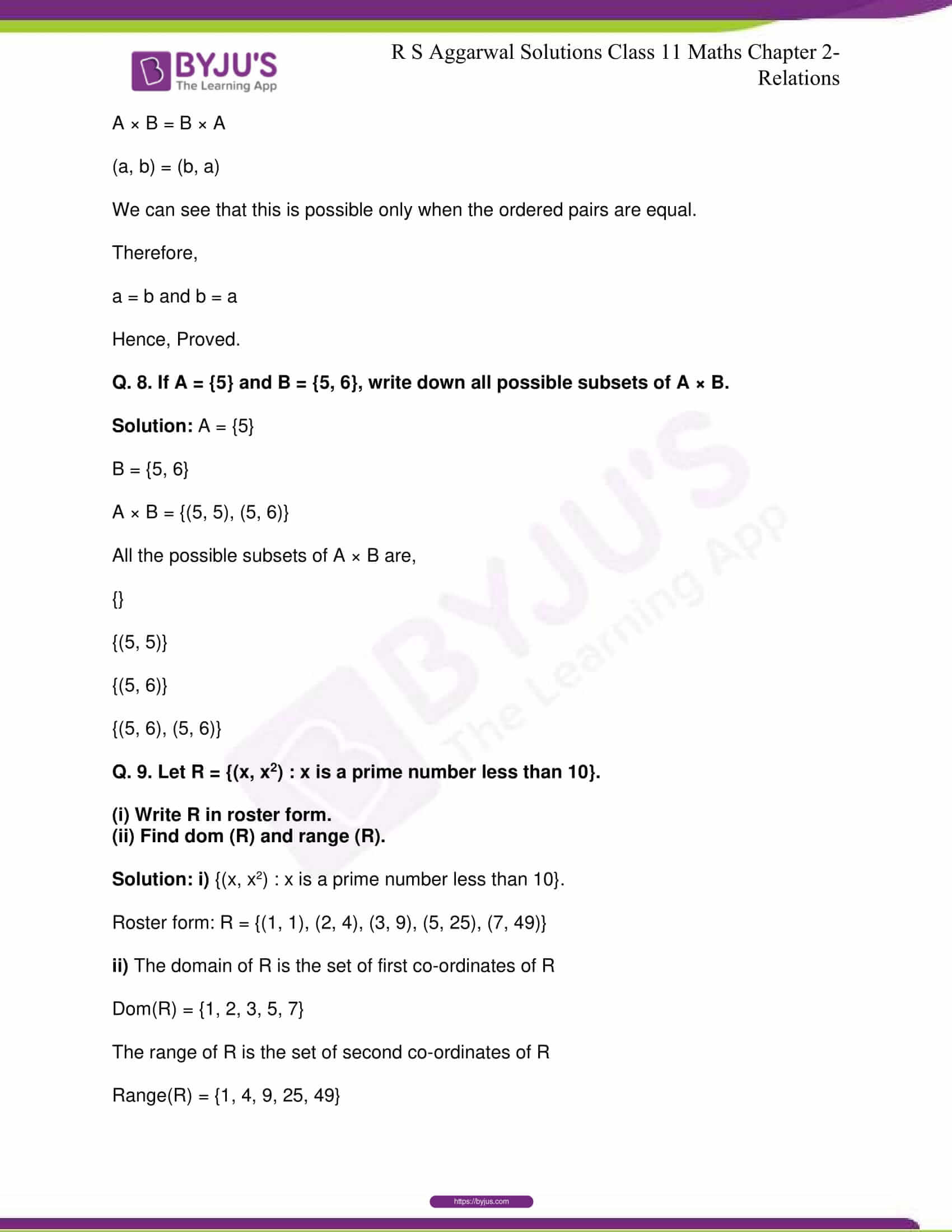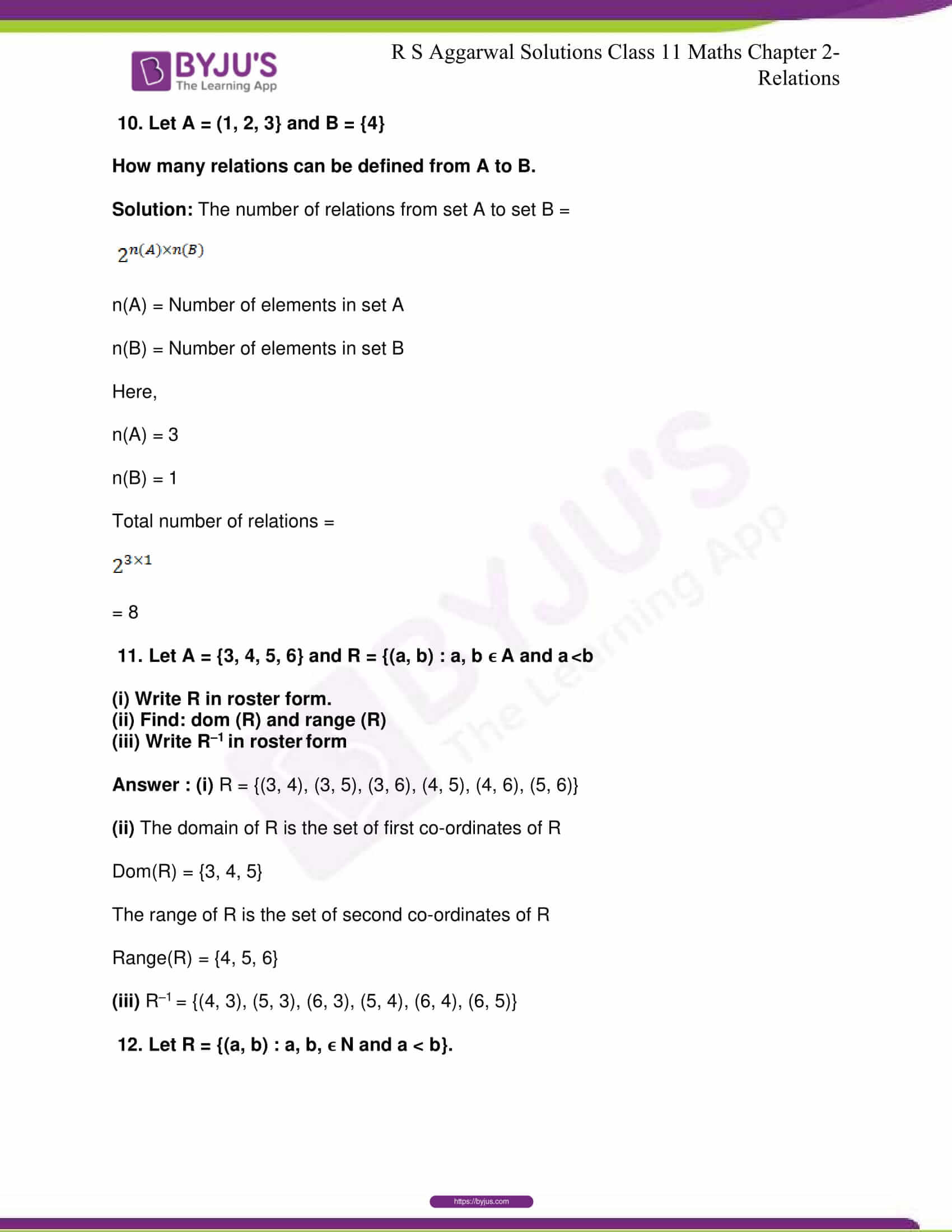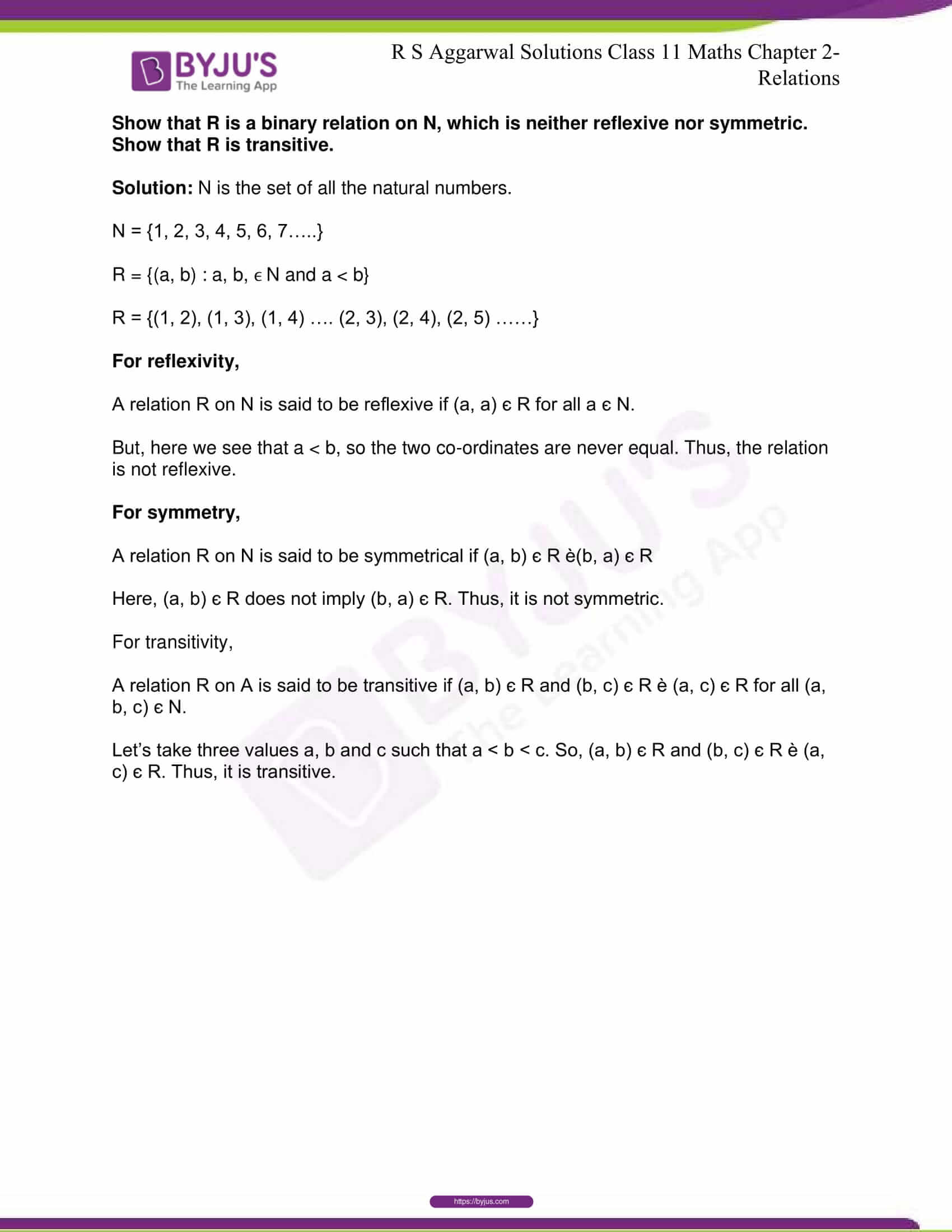## R S Aggarwal Solutions for Class 11 Maths Chapter 2- Relations

Chapter 2, Relations, is composed of 5 exercises and the solutions given here contain answers to all the questions present in this exercise. Let us have a look at some of the topics that are being discussed in this chapter.

2.1 Ordered pair

2.2 Cartesian product of two sets

2.3 Relations

2.4 Domain, Range and Co-domain of a relation

2.5 Inverse Relation

2.6 Equivalence Relation

## R S Aggarwal Solutions for Class 11 Maths Chapter 2- Relations

Let A and B be two non-empty sets. Then, a relation R from A to B is a subset of A x B. The chapter also deals with the explanation and problems of different concepts related to Relations. Read and learn Chapter 2 of R S Aggarwal textbook to familiarize with the concepts related to Relations. Learn the R S Aggarwal Solutions for Class 11 effectively to score high in the examination.